• Размер: 3.9 Mегабайта
• Количество слайдов: 84

## Описание презентации The 14 th of JANUARY CHAPTER: ELECTRICITY по слайдам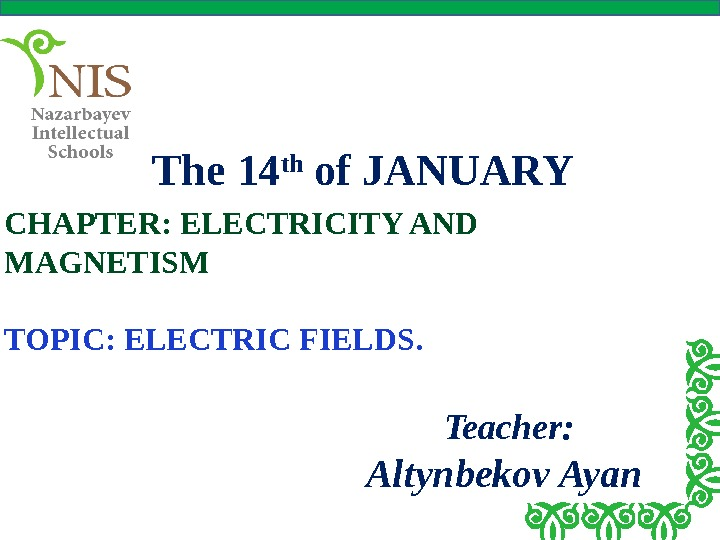The 14 th of JANUARY CHAPTER: ELECTRICITY AND MAGNETISM TOPIC: ELECTRIC FIELDS. Teacher: Altynbekov Ayan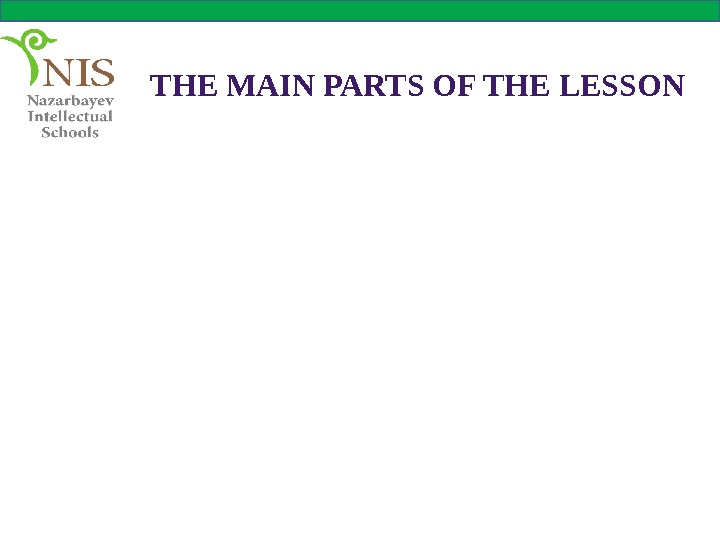THE MAIN PARTS OF THE LESSON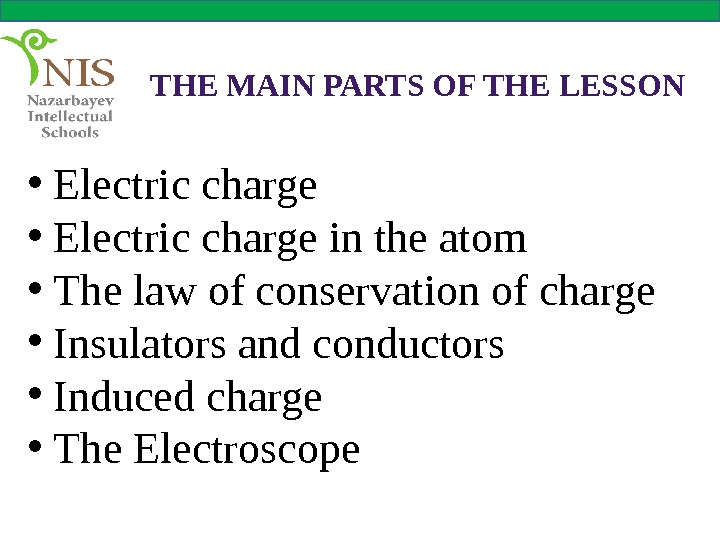THE MAIN PARTS OF THE LESSON • Electric charge in the atom • The law of conservation of charge • Insulators and conductors • Induced charge • The Electroscope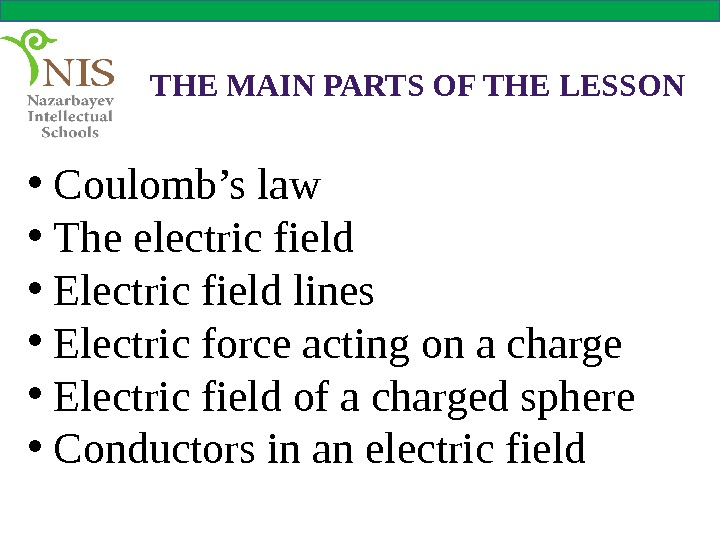THE MAIN PARTS OF THE LESSON • Coulomb’s law • The electric field • Electric field lines • Electric force acting on a charge • Electric field of a charged sphere • Conductors in an electric field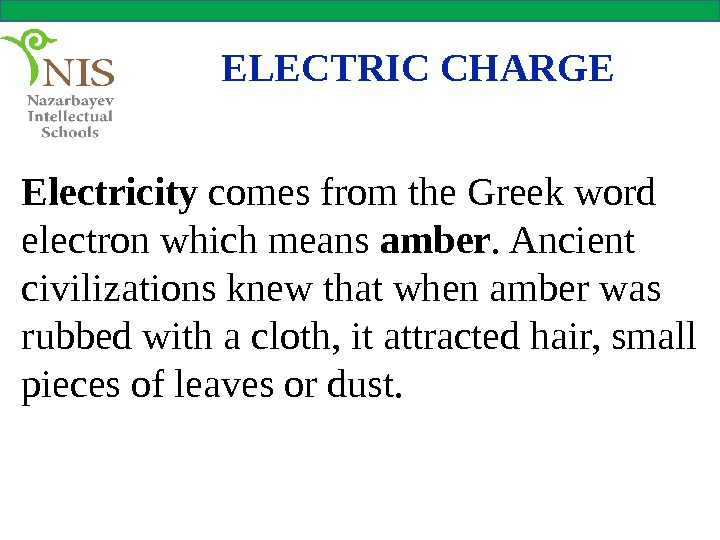ELECTRIC CHARGE Electricity comes from the Greek word electron which means amber. Ancient civilizations knew that when amber was rubbed with a cloth, it attracted hair, small pieces of leaves or dust.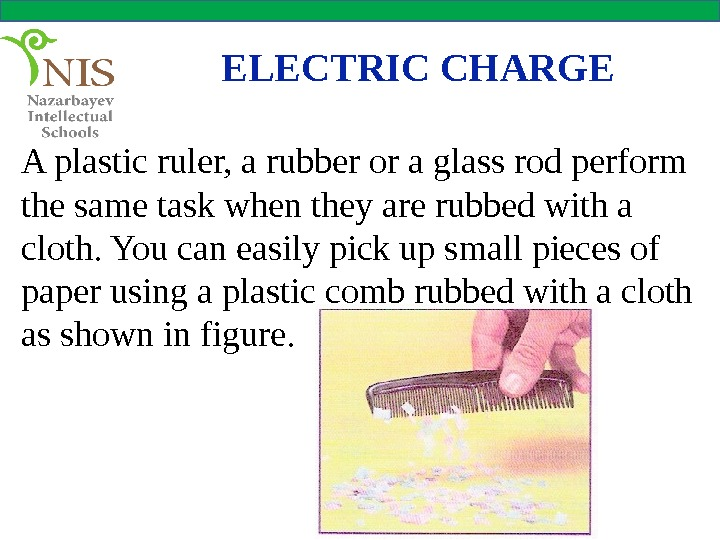A plastic ruler, a rubber or a glass rod perform the same task when they are rubbed with a cloth. You can easily pick up small pieces of paper using a plastic comb rubbed with a cloth as shown in figure. ELECTRIC CHARG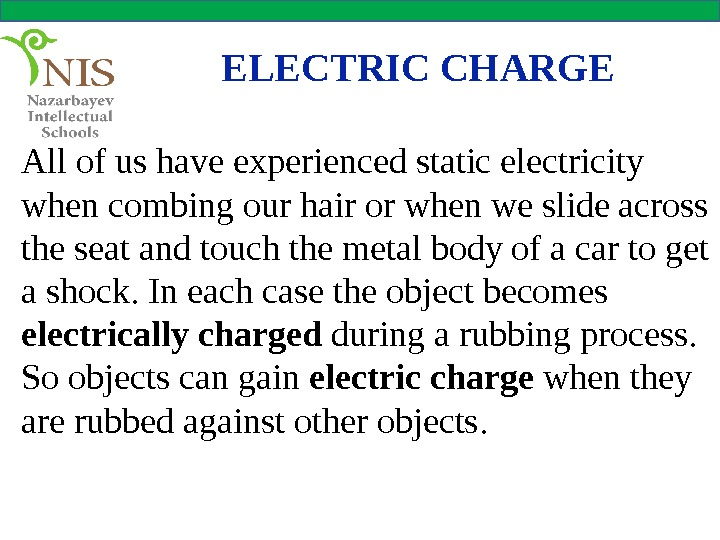ELECTRIC CHARGE All of us have experienced static electricity when combing our hair or when we slide across the seat and touch the metal body of a car to get a shock. In each case the object becomes electrically charged during a rubbing process. So objects can gain electric charge when they are rubbed against other objects.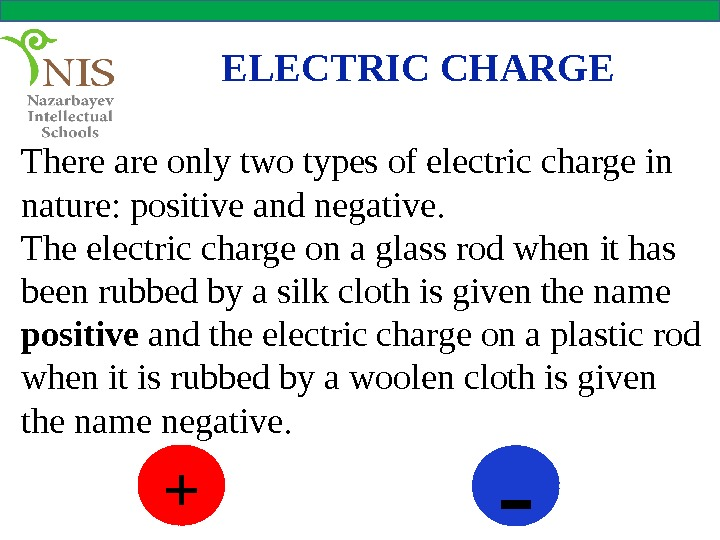ELECTRIC CHARGE There are only two types of electric charge in nature: positive and negative. The electric charge on a glass rod when it has been rubbed by a silk cloth is given the name positive and the electric charge on a plastic rod when it is rubbed by a woolen cloth is given the name negative. + —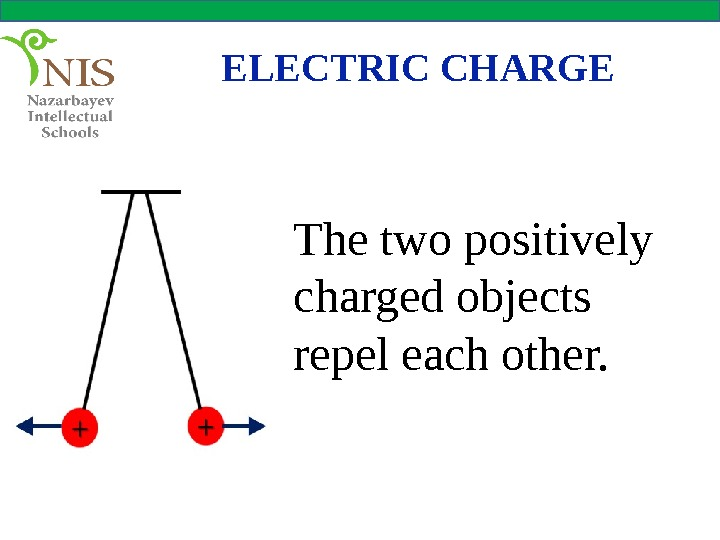ELECTRIC CHARGE The two positively charged objects repel each other.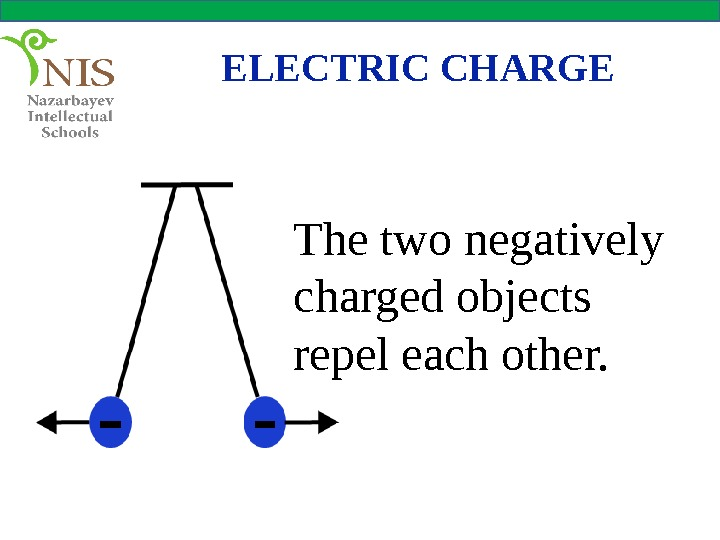ELECTRIC CHARGE The two negatively charged objects repel each other.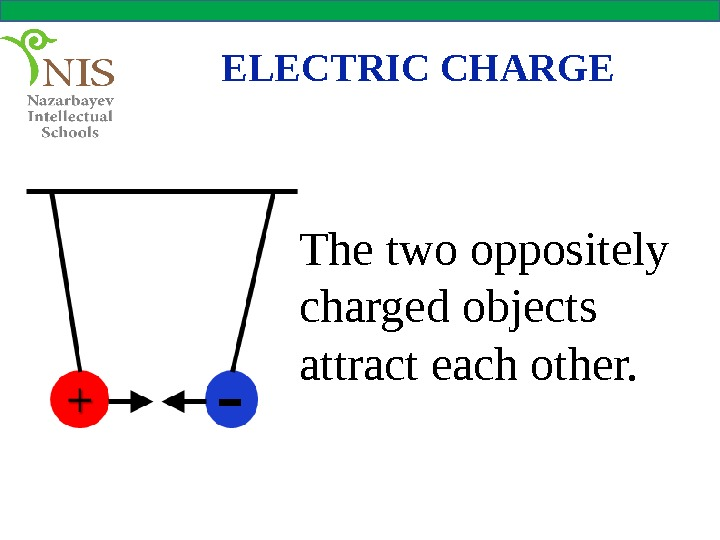ELECTRIC CHARGE The two oppositely charged objects attract each other.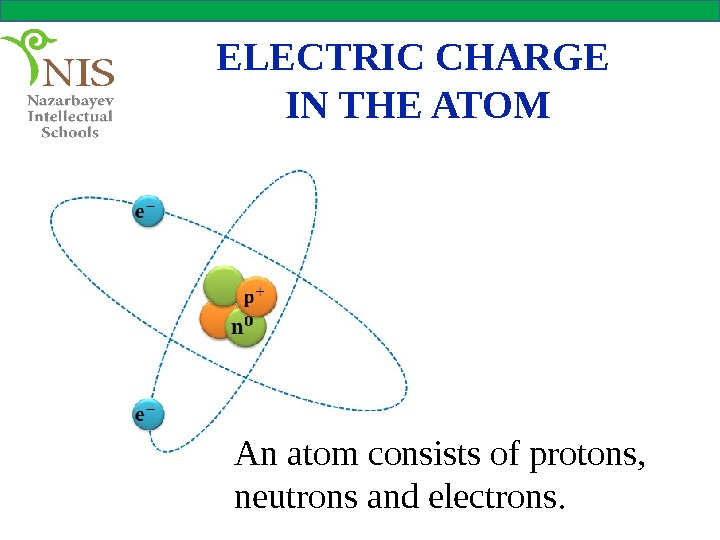ELECTRIC CHARGE IN THE ATOM An atom consists of protons, neutrons and electrons.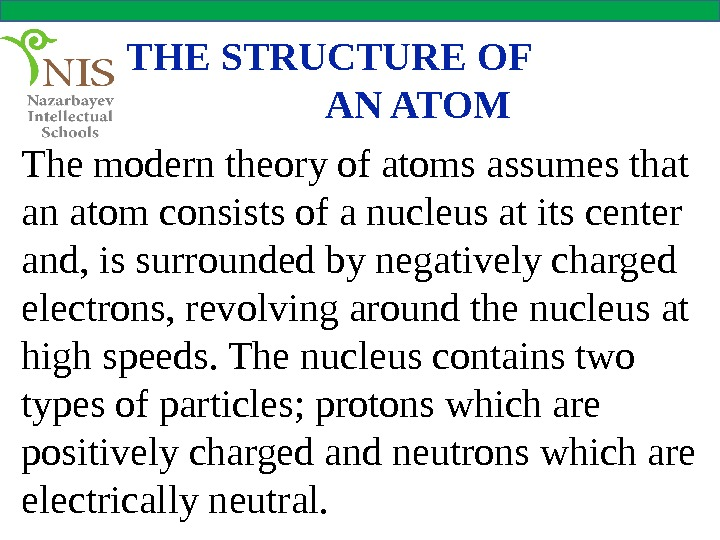THE STRUCTURE OF AN ATOM The modern theory of atoms assumes that an atom consists of a nucleus at its center and, is surrounded by negatively charged electrons, revolving around the nucleus at high speeds. The nucleus contains two types of particles; protons which are positively charged and neutrons which are electrically neutral.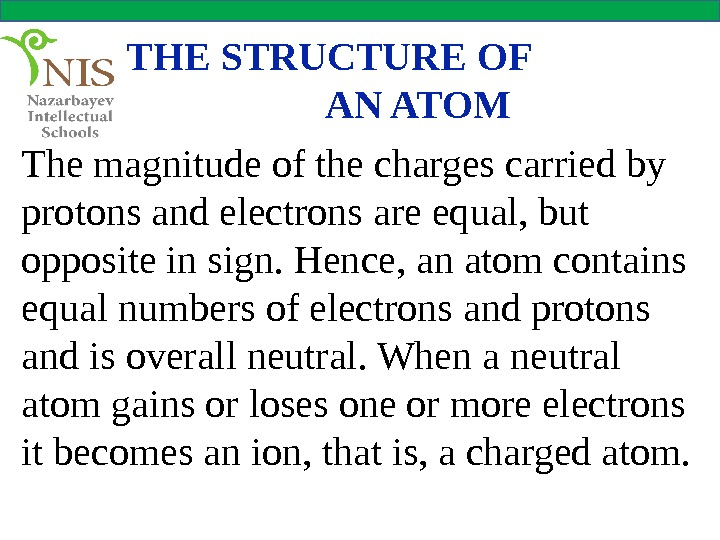THE STRUCTURE OF AN ATOM The magnitude of the charges carried by protons and electrons are equal, but opposite in sign. Hence, an atom contains equal numbers of electrons and protons and is overall neutral. When a neutral atom gains or loses one or more electrons it becomes an ion, that is, a charged atom.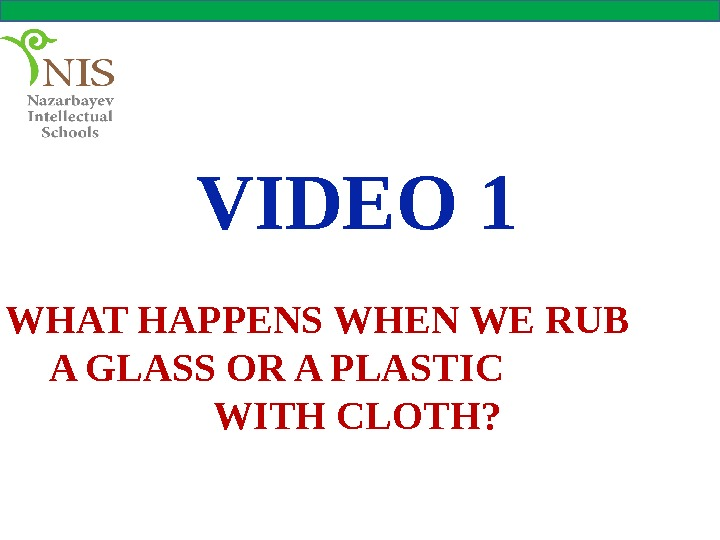VIDEO 1 WHAT HAPPENS WHEN WE RUB A GLASS OR A PLASTIC WITH CLOTH?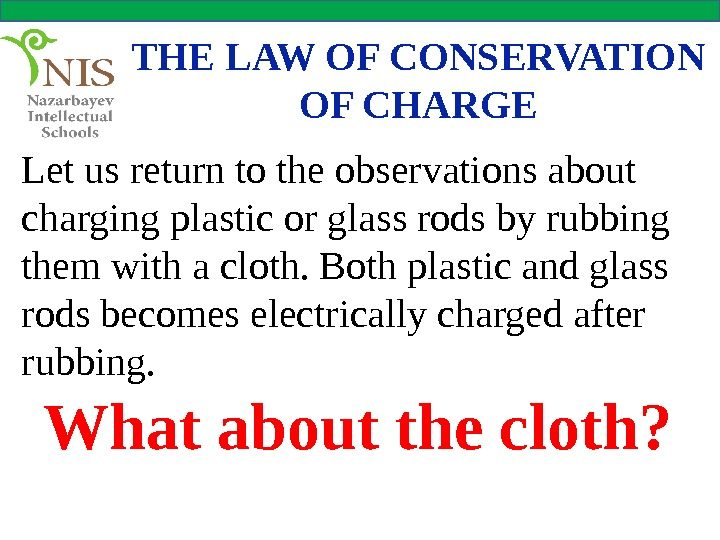THE LAW OF CONSERVATION OF CHARGE Let us return to the observations about charging plastic or glass rods by rubbing them with a cloth. Both plastic and glass rods becomes electrically charged after rubbing. What about the cloth?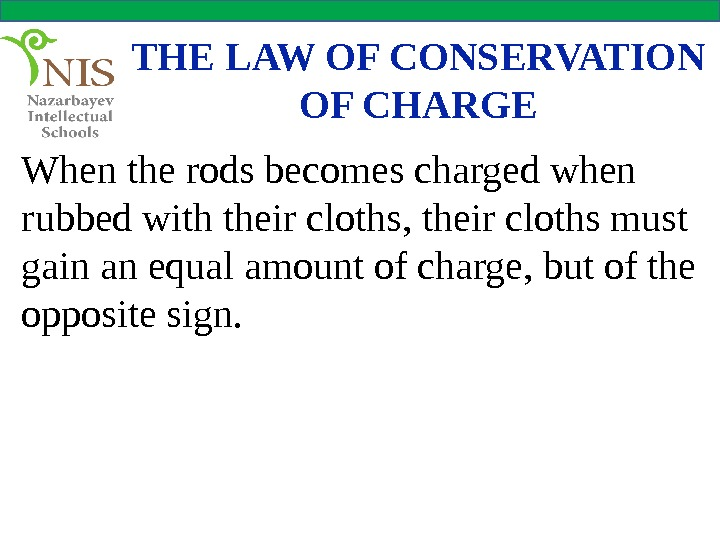THE LAW OF CONSERVATION OF CHARGE When the rods becomes charged when rubbed with their cloths, their cloths must gain an equal amount of charge, but of the opposite sign.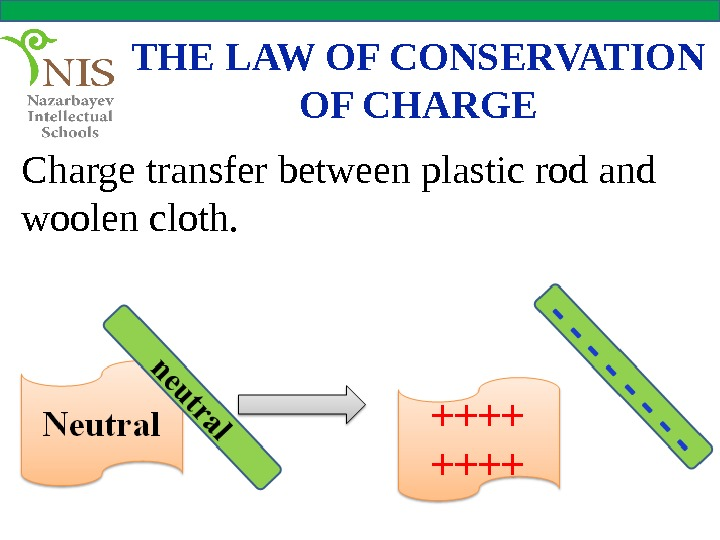THE LAW OF CONSERVATION OF CHARGE Charge transfer between plastic rod and woolen cloth.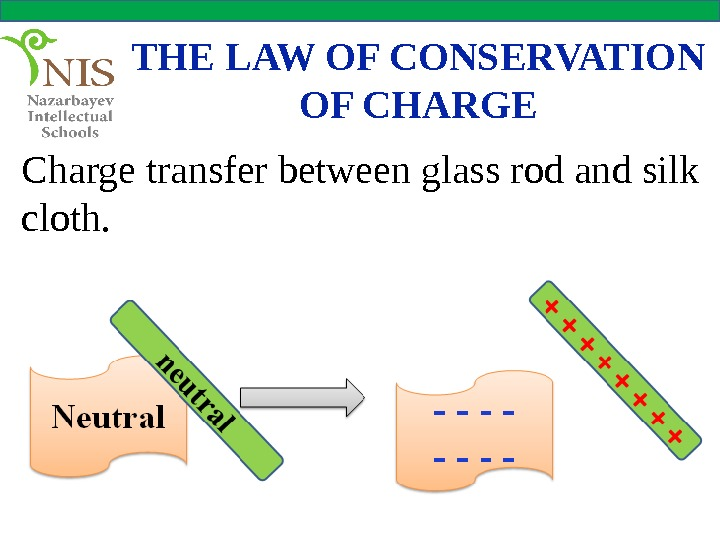THE LAW OF CONSERVATION OF CHARGE Charge transfer between glass rod and silk cloth.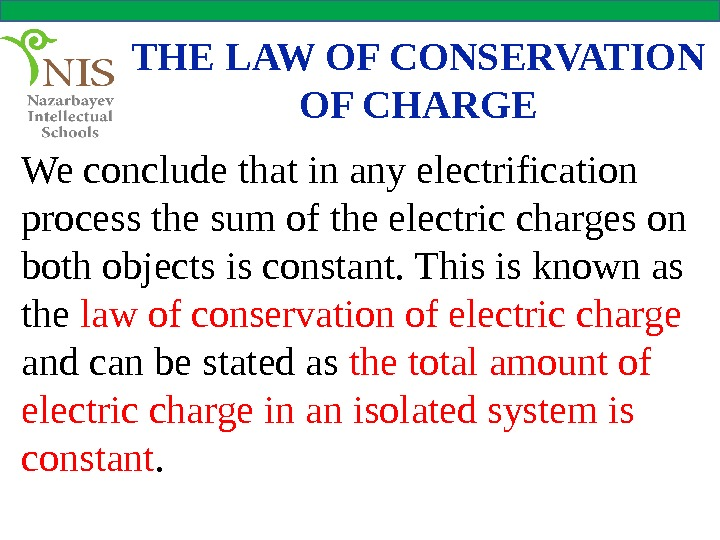THE LAW OF CONSERVATION OF CHARGE We conclude that in any electrification process the sum of the electric charges on both objects is constant. This is known as the law of conservation of electric charge and can be stated as the total amount of electric charge in an isolated system is constant.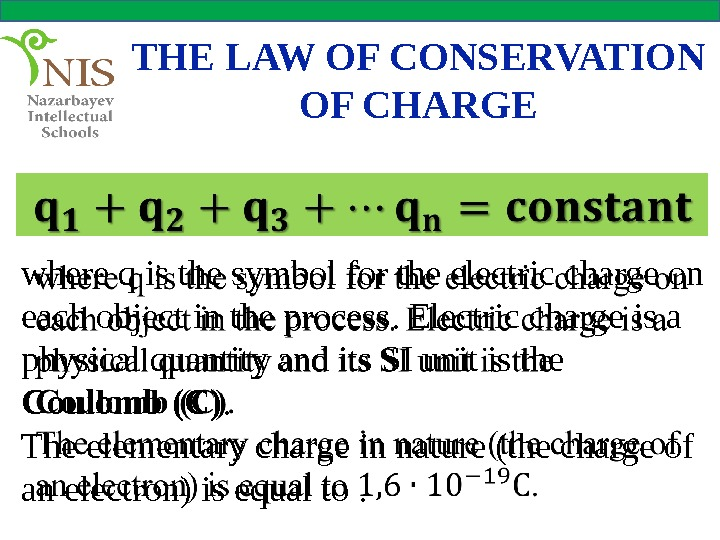THE LAW OF CONSERVATION OF CHARGE where q is the symbol for the electric charge on each object in the process. Electric charge is a physical quantity and its SI unit is the Coulomb (C). The elementary charge in nature (the charge of an electron) is equal to.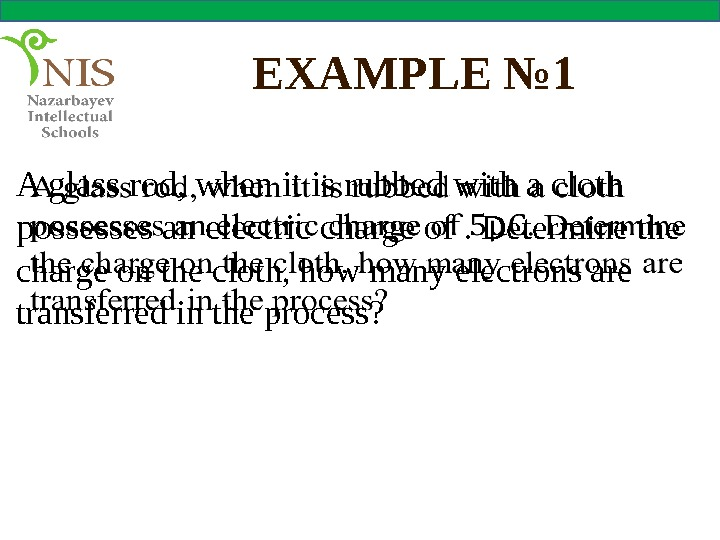EXAMPLE № 1 A glass rod, when it is rubbed with a cloth possesses an electric charge of . Determine the charge on the cloth, how many electrons are transferred in the process?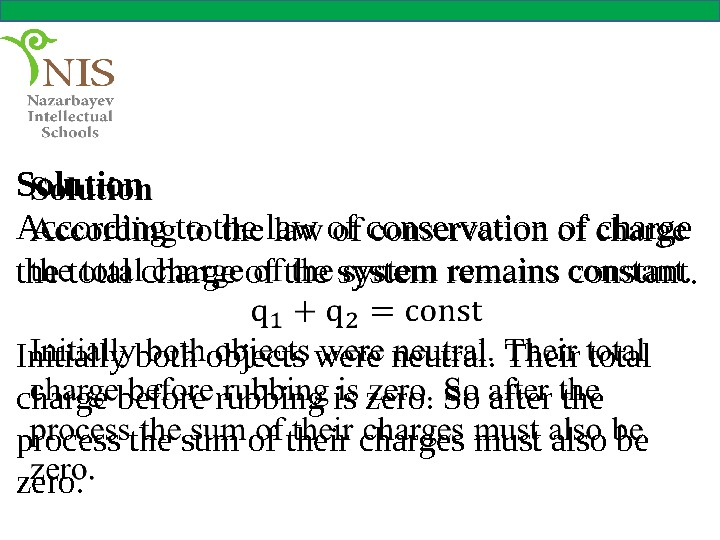Solution According to the law of conservation of charge the total charge of the system remains constant. Initially both objects were neutral. Their total charge before rubbing is zero. So after the process the sum of their charges must also be zero.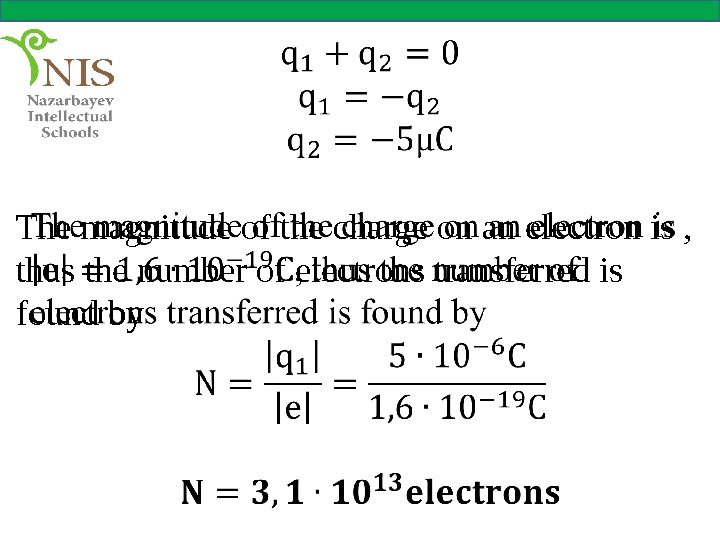The magnitude of the charge on an electron is , thus the number of electrons transferred is found by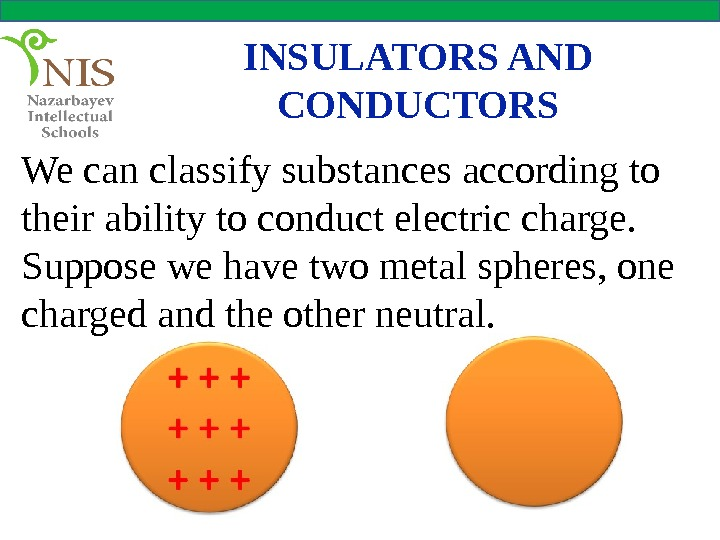INSULATORS AND CONDUCTORS We can classify substances according to their ability to conduct electric charge. Suppose we have two metal spheres, one charged and the other neutral.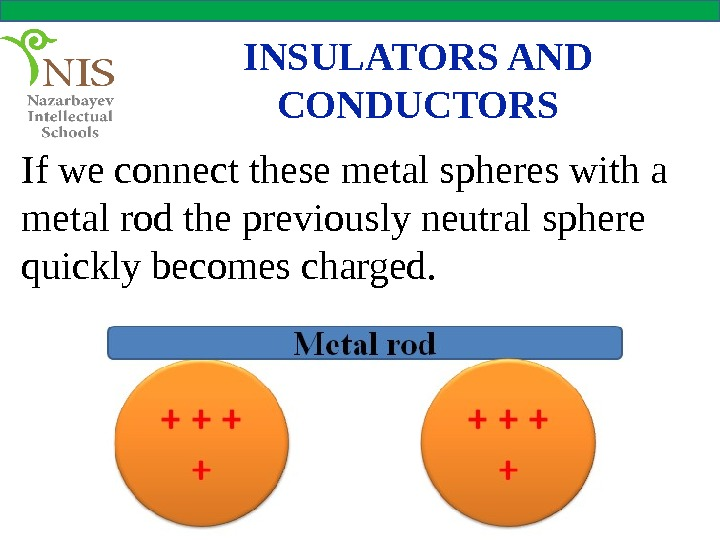INSULATORS AND CONDUCTORS If we connect these metal spheres with a metal rod the previously neutral sphere quickly becomes charged.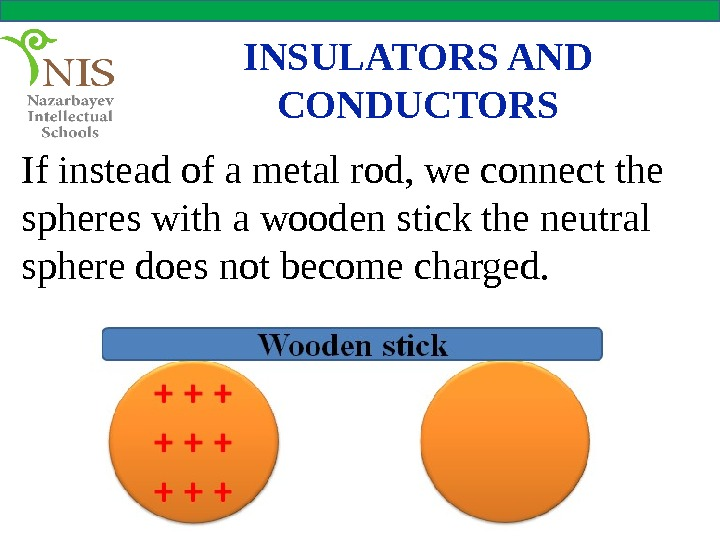INSULATORS AND CONDUCTORS If instead of a metal rod, we connect the spheres with a wooden stick the neutral sphere does not become charged.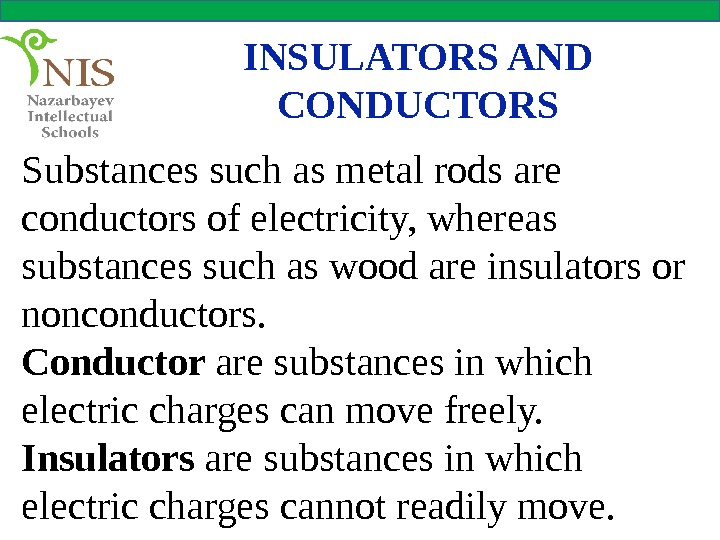INSULATORS AND CONDUCTORS Substances such as metal rods are conductors of electricity, whereas substances such as wood are insulators or nonconductors. Conductor are substances in which electric charges can move freely. Insulators are substances in which electric charges cannot readily move.VIDEO 2 CONDUCTORS AND INSULATORS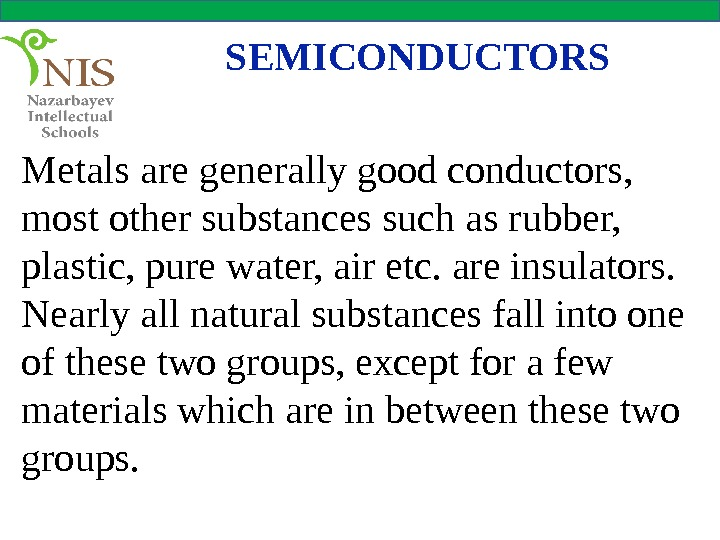SEMICONDUCTORS Metals are generally good conductors, most other substances such as rubber, plastic, pure water, air etc. are insulators. Nearly all natural substances fall into one of these two groups, except for a few materials which are in between these two groups.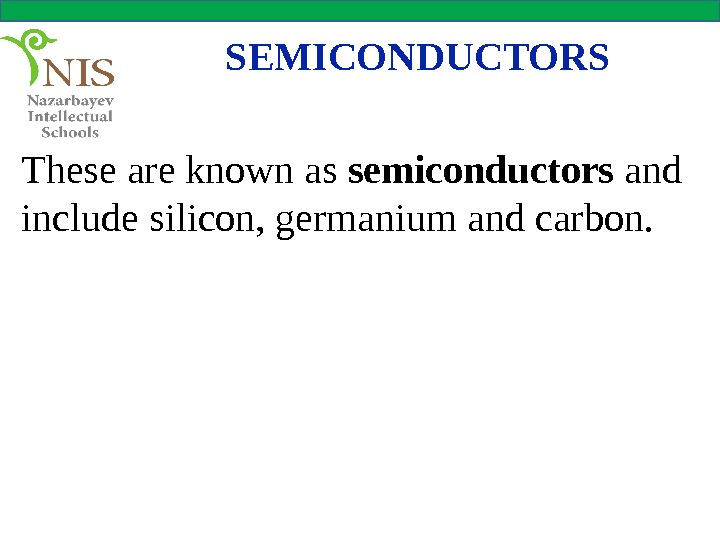SEMICONDUCTORS These are known as semiconductors and include silicon, germanium and carbon.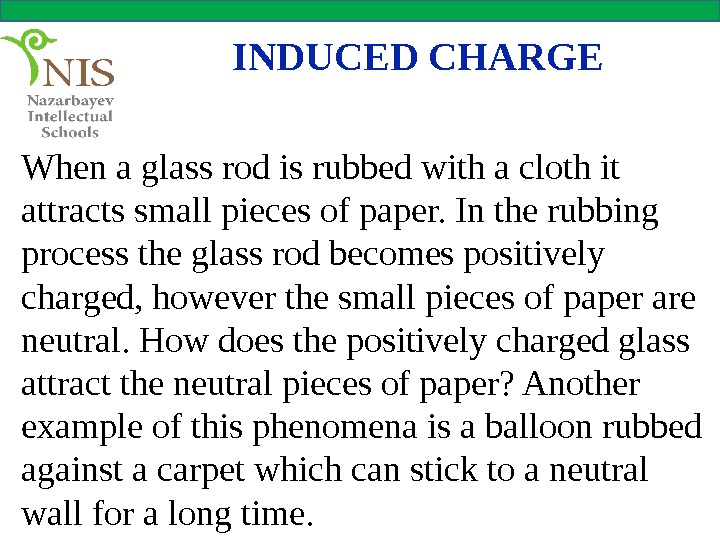INDUCED CHARGE When a glass rod is rubbed with a cloth it attracts small pieces of paper. In the rubbing process the glass rod becomes positively charged, however the small pieces of paper are neutral. How does the positively charged glass attract the neutral pieces of paper? Another example of this phenomena is a balloon rubbed against a carpet which can stick to a neutral wall for a long time.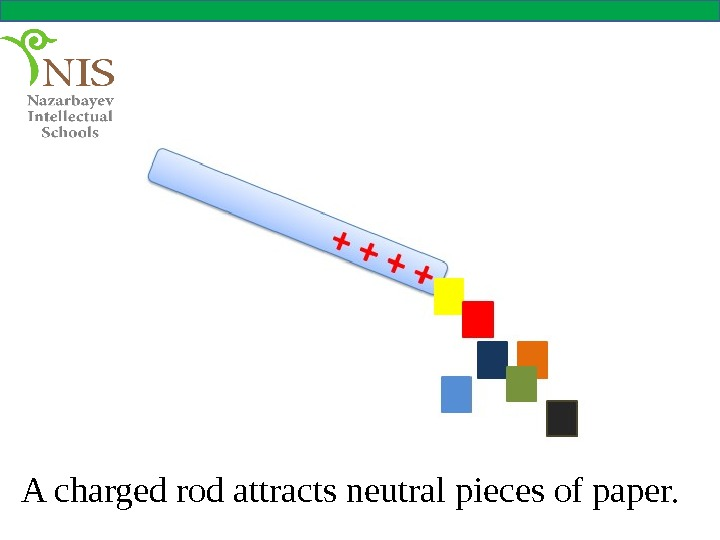A charged rod attracts neutral pieces of paper.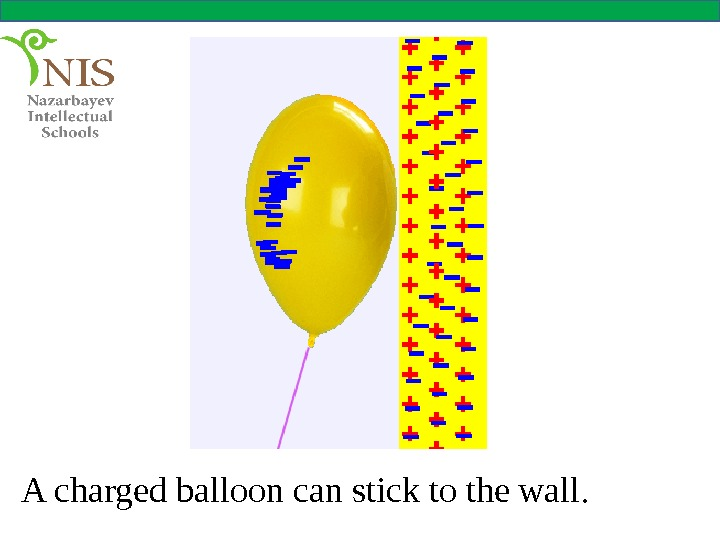A charged balloon can stick to the wall.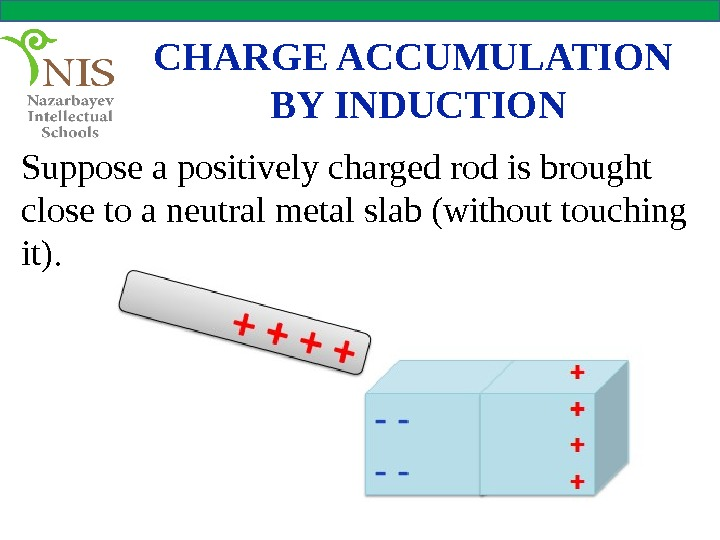CHARGE ACCUMULATION BY INDUCTION Suppose a positively charged rod is brought close to a neutral metal slab (without touching it).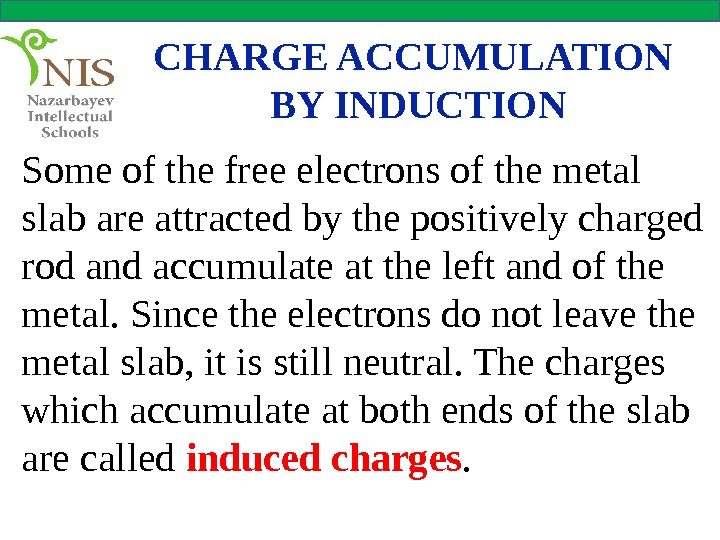CHARGE ACCUMULATION BY INDUCTION Some of the free electrons of the metal slab are attracted by the positively charged rod and accumulate at the left and of the metal. Since the electrons do not leave the metal slab, it is still neutral. The charges which accumulate at both ends of the slab are called induced charges.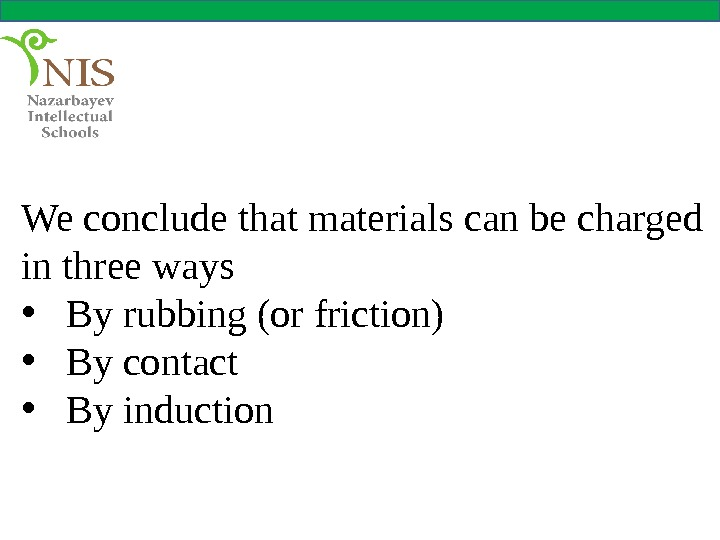We conclude that materials can be charged in three ways • By rubbing (or friction) • By contact • By induction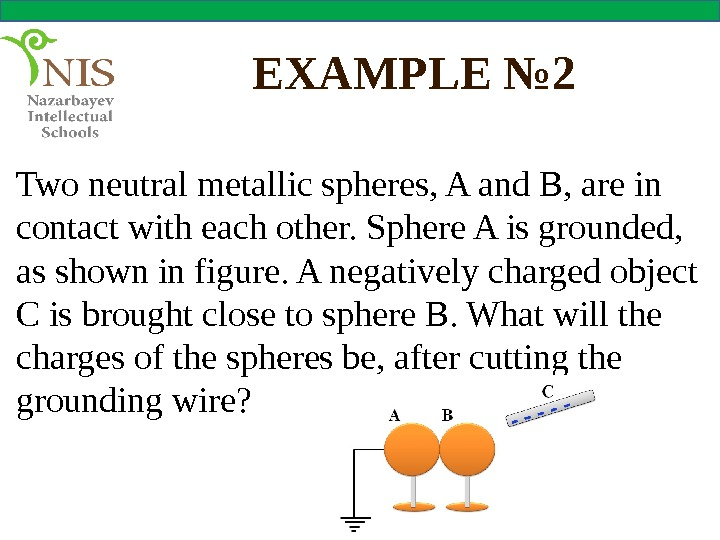EXAMPLE № 2 Two neutral metallic spheres, A and B, are in contact with each other. Sphere A is grounded, as shown in figure. A negatively charged object C is brought close to sphere B. What will the charges of the spheres be, after cutting the grounding wire?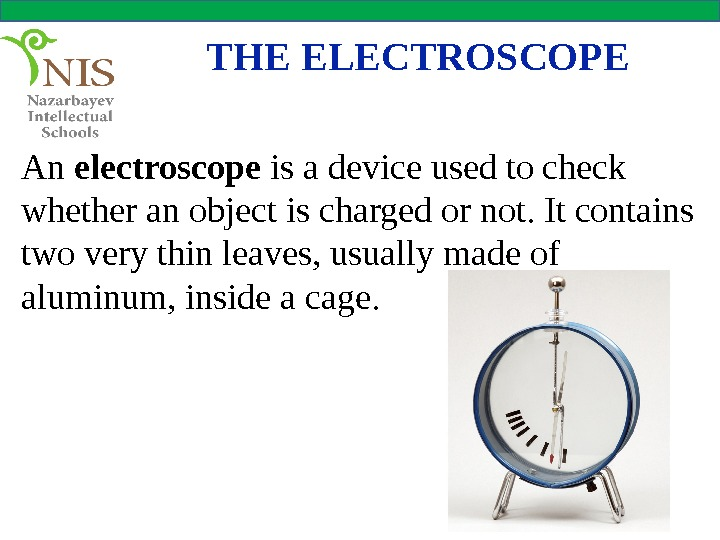THE ELECTROSCOPE An electroscope is a device used to check whether an object is charged or not. It contains two very thin leaves, usually made of aluminum, inside a cage.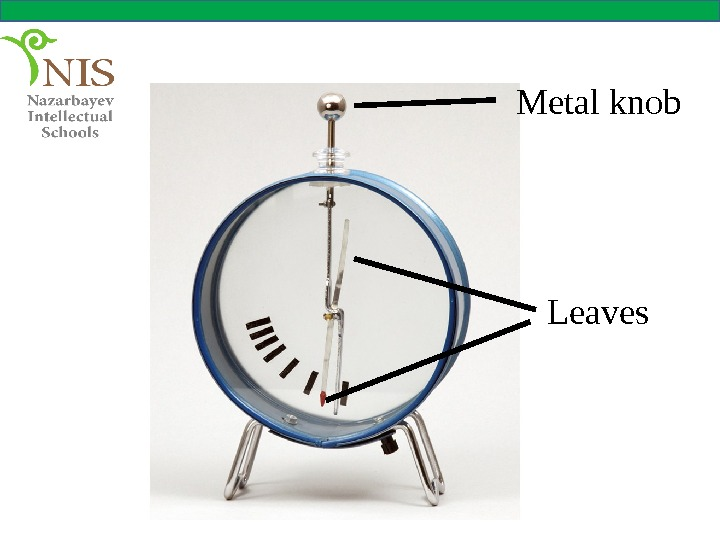Metal knob Leaves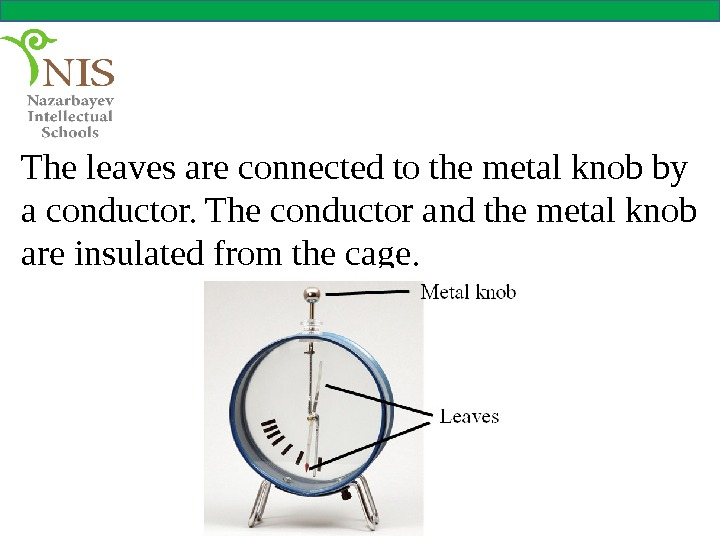The leaves are connected to the metal knob by a conductor. The conductor and the metal knob are insulated from the cage.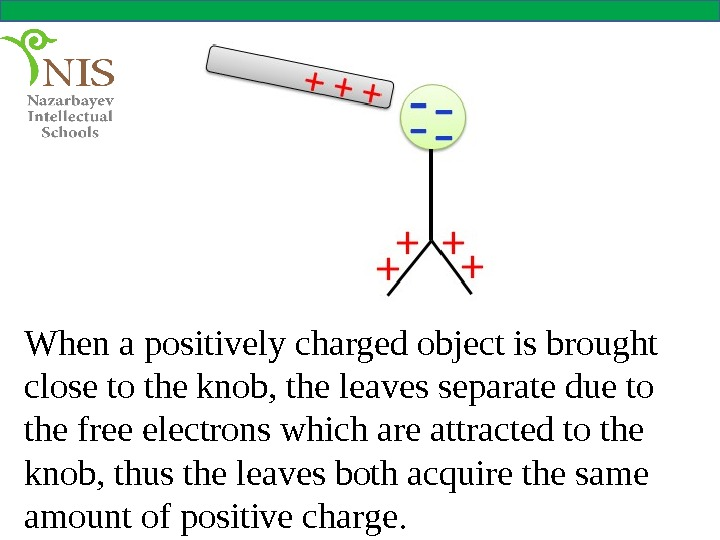When a positively charged object is brought close to the knob, the leaves separate due to the free electrons which are attracted to the knob, thus the leaves both acquire the same amount of positive charge.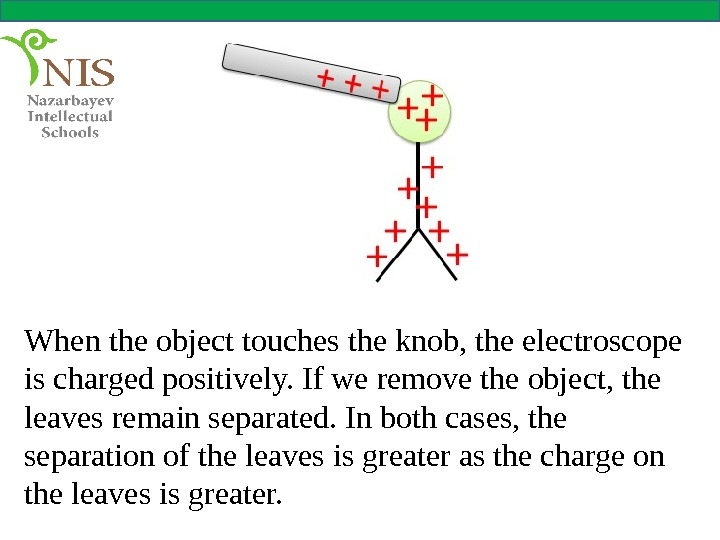When the object touches the knob, the electroscope is charged positively. If we remove the object, the leaves remain separated. In both cases, the separation of the leaves is greater as the charge on the leaves is greater.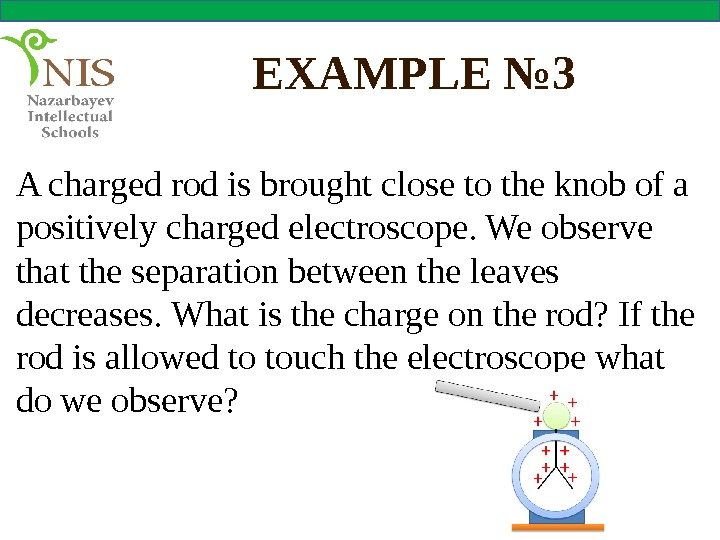EXAMPLE № 3 A charged rod is brought close to the knob of a positively charged electroscope. We observe that the separation between the leaves decreases. What is the charge on the rod? If the rod is allowed to touch the electroscope what do we observe?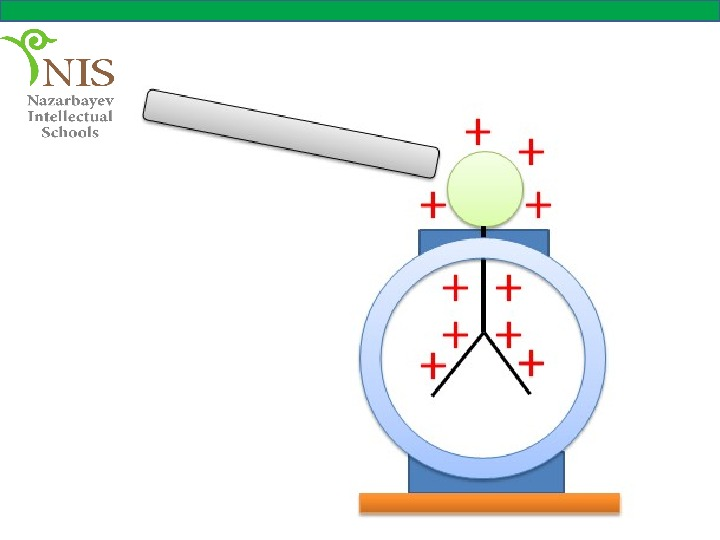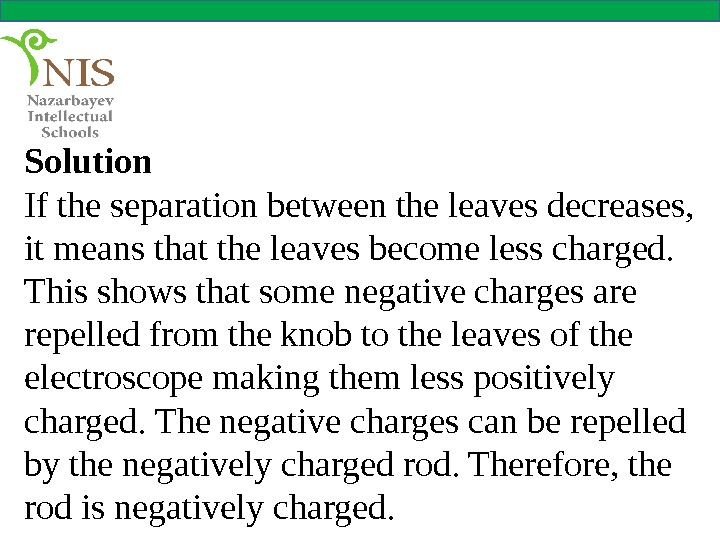Solution If the separation between the leaves decreases, it means that the leaves become less charged. This shows that some negative charges are repelled from the knob to the leaves of the electroscope making them less positively charged. The negative charges can be repelled by the negatively charged rod. Therefore, the rod is negatively charged.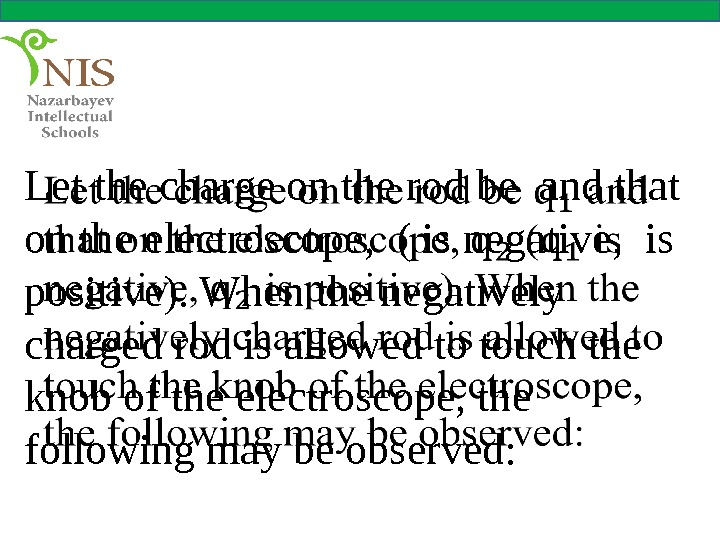Let the charge on the rod be and that on the electroscope, ( is negative, is positive). When the negatively charged rod is allowed to touch the knob of the electroscope, the following may be observed: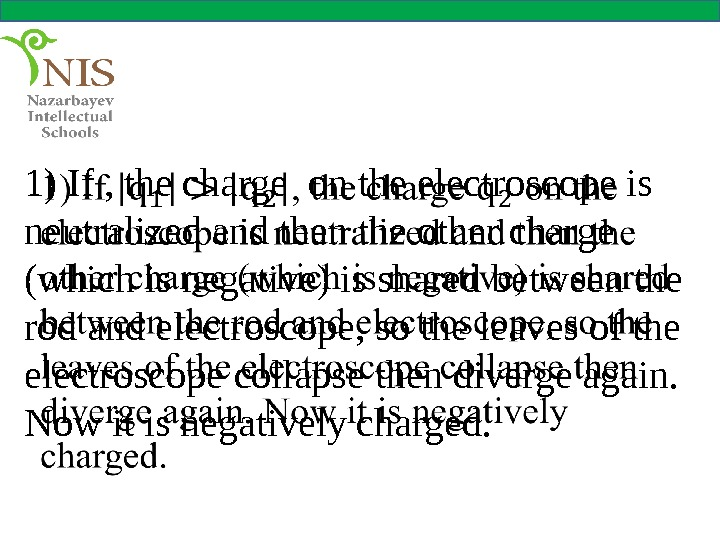1) If , the charge on the electroscope is neutralized and then the other charge (which is negative) is shared between the rod and electroscope, so the leaves of the electroscope collapse then diverge again. Now it is negatively charged.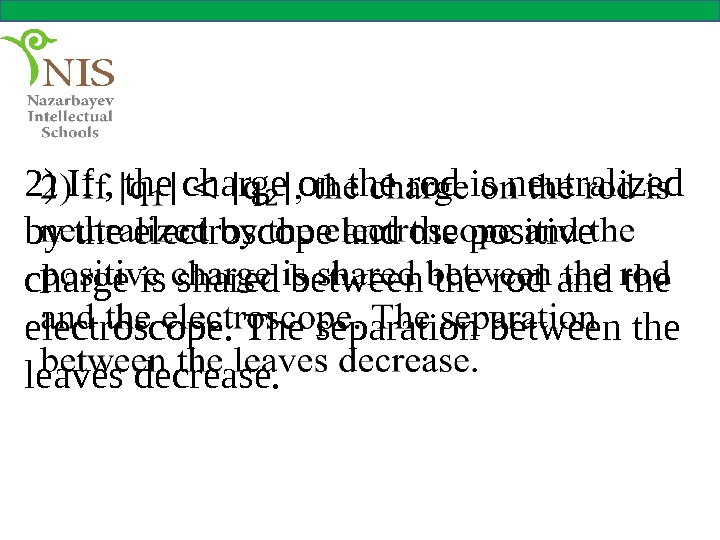2) If , the charge on the rod is neutralized by the electroscope and the positive charge is shared between the rod and the electroscope. The separation between the leaves decrease.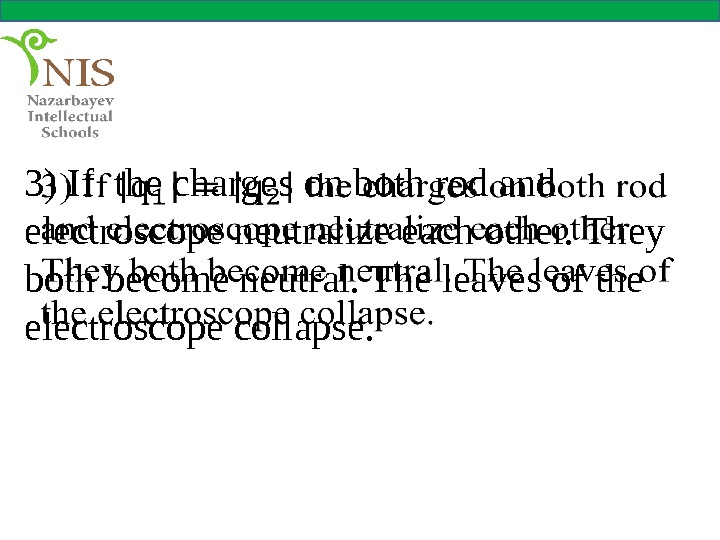3) If the charges on both rod and electroscope neutralize each other. They both become neutral. The leaves of the electroscope collapse.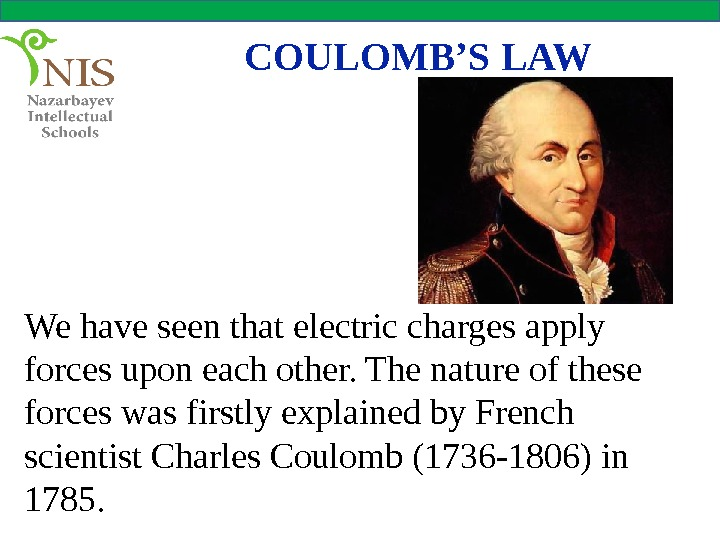COULOMB’S LAW We have seen that electric charges apply forces upon each other. The nature of these forces was firstly explained by French scientist Charles Coulomb (1736 -1806) in 1785.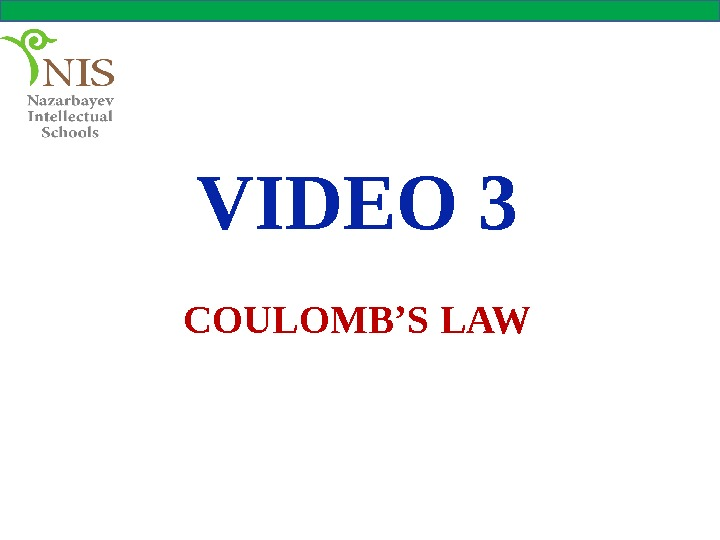VIDEO 3 COULOMB’S LAW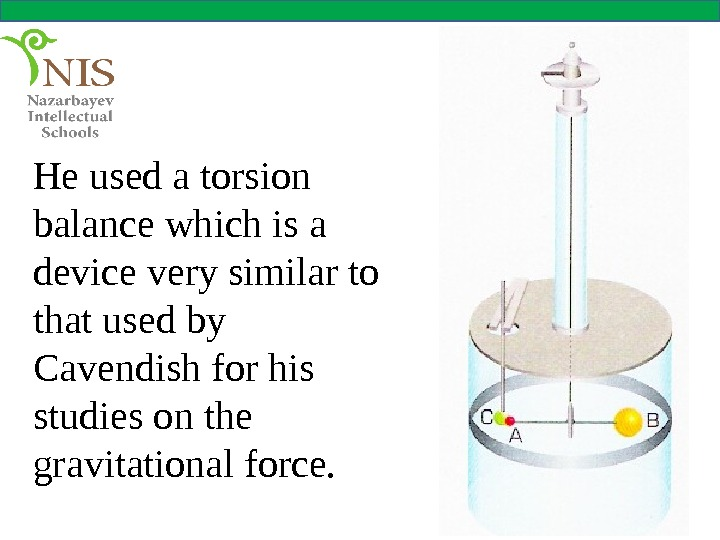He used a torsion balance which is a device very similar to that used by Cavendish for his studies on the gravitational force.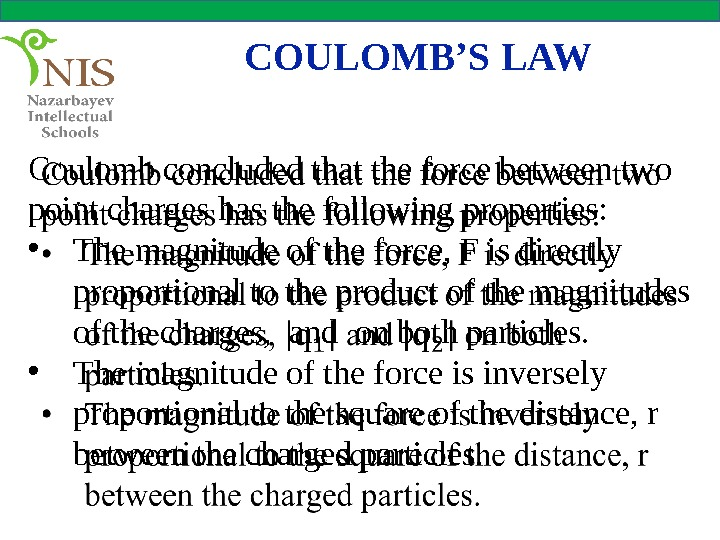COULOMB’S LAW Coulomb concluded that the force between two point charges has the following properties: • The magnitude of the force, F is directly proportional to the product of the magnitudes of the charges, and on both particles. • The magnitude of the force is inversely proportional to the square of the distance, r between the charged particles.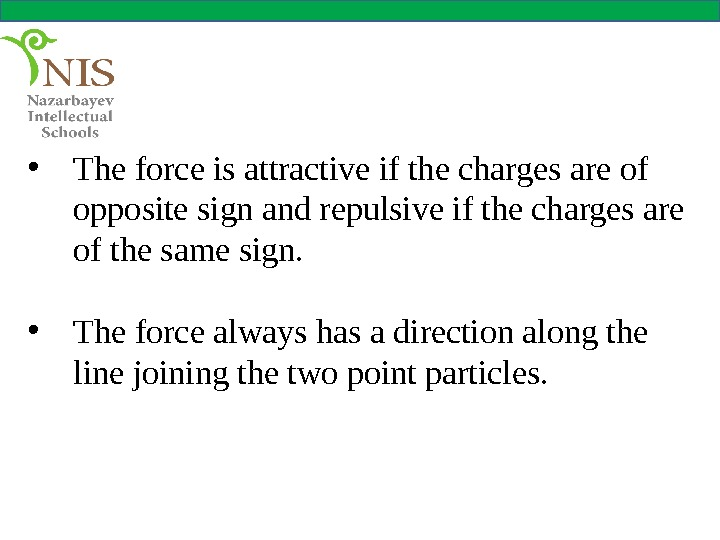• The force is attractive if the charges are of opposite sign and repulsive if the charges are of the same sign. • The force always has a direction along the line joining the two point particles.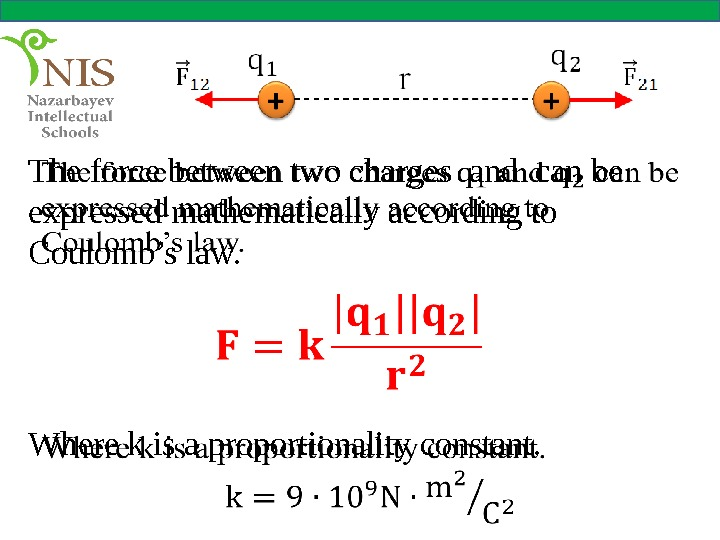The force between two charges and can be expressed mathematically according to Coulomb’s law. Where k is a proportionality constant.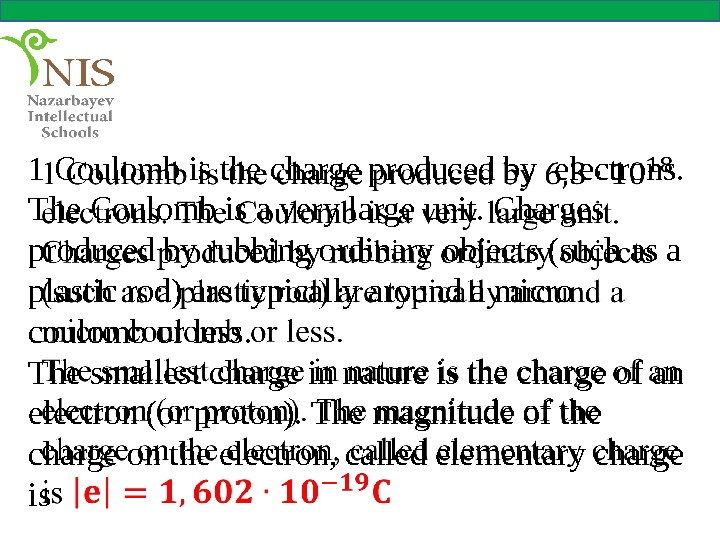1 Coulomb is the charge produced by electrons. The Coulomb is a very large unit. Charges produced by rubbing ordinary objects (such as a plastic rod) are typically around a micro coulomb or less. The smallest charge in nature is the charge of an electron (or proton). The magnitude of the charge on the electron, called elementary charge is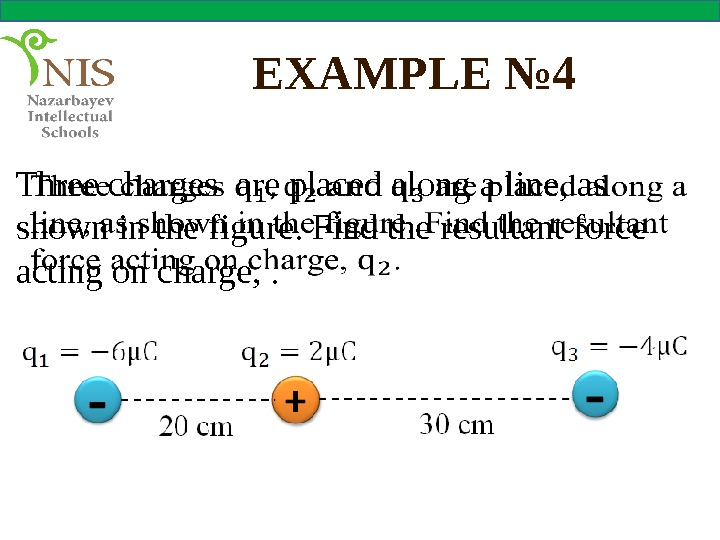EXAMPLE № 4 Three charges are placed along a line, as shown in the figure. Find the resultant force acting on charge, .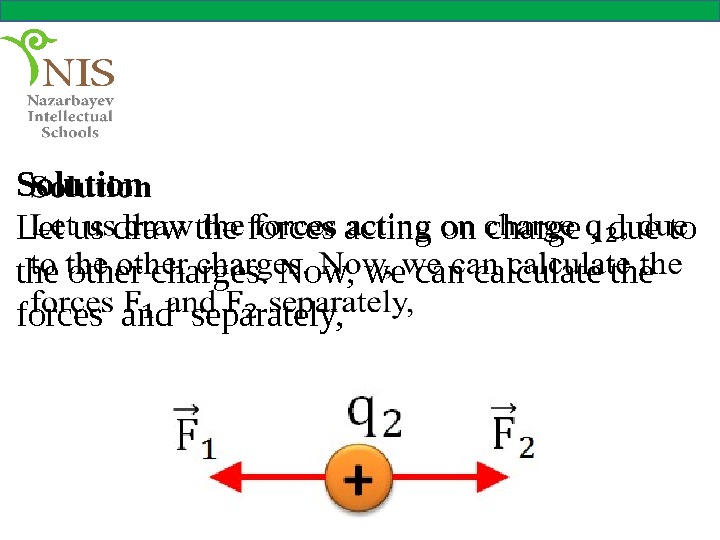Solution Let us draw the forces acting on charge , due to the other charges. Now, we can calculate the forces and separately,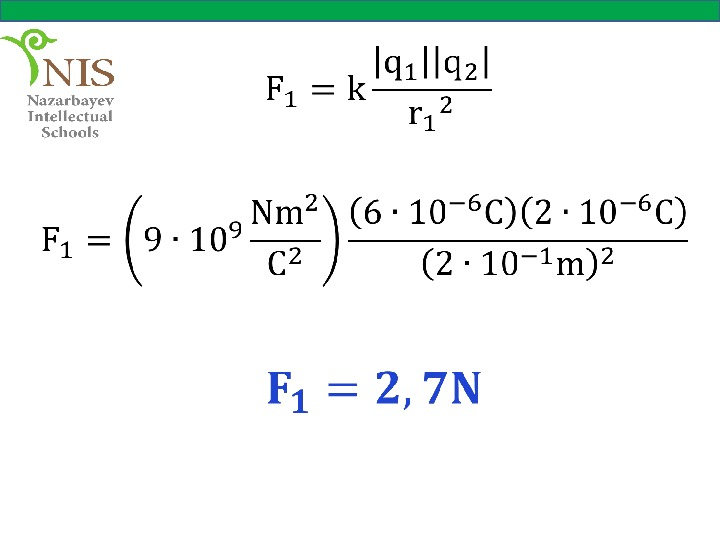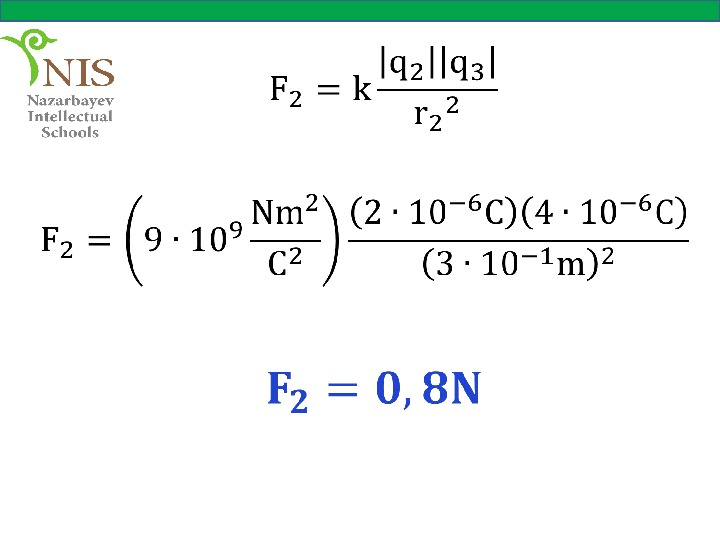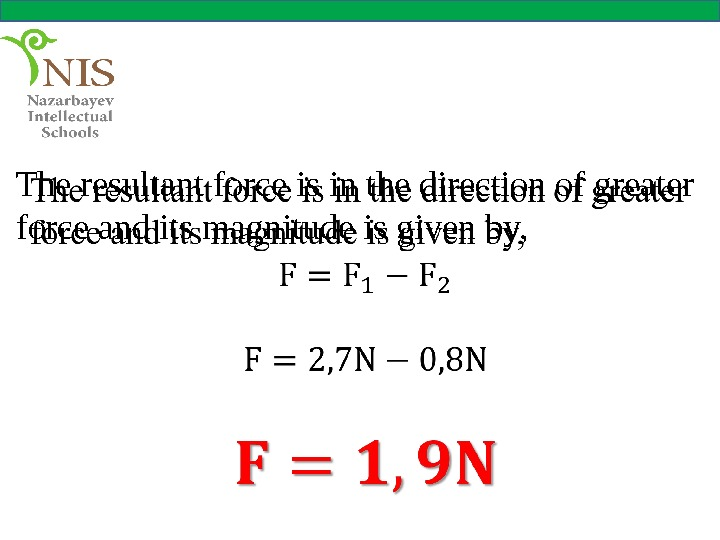The resultant force is in the direction of greater force and its magnitude is given by,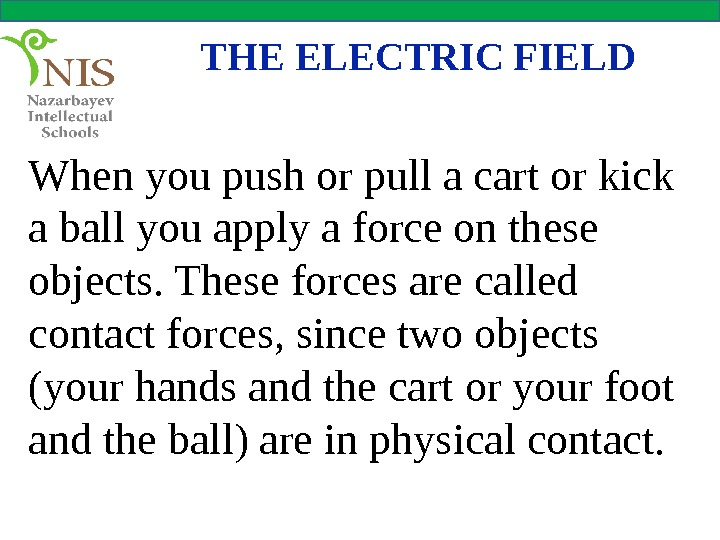THE ELECTRIC FIELD When you push or pull a cart or kick a ball you apply a force on these objects. These forces are called contact forces, since two objects (your hands and the cart or your foot and the ball) are in physical contact.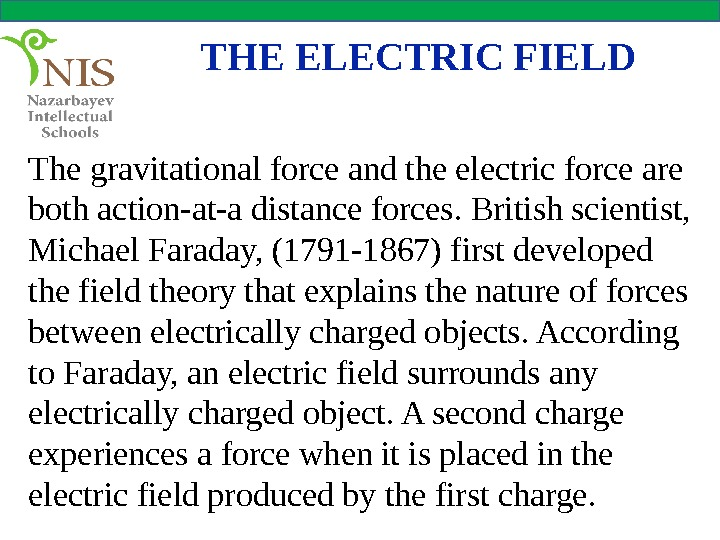THE ELECTRIC FIELD The gravitational force and the electric force are both action-at-a distance forces. British scientist, Michael Faraday, (1791 -1867) first developed the field theory that explains the nature of forces between electrically charged objects. According to Faraday, an electric field surrounds any electrically charged object. A second charge experiences a force when it is placed in the electric field produced by the first charge.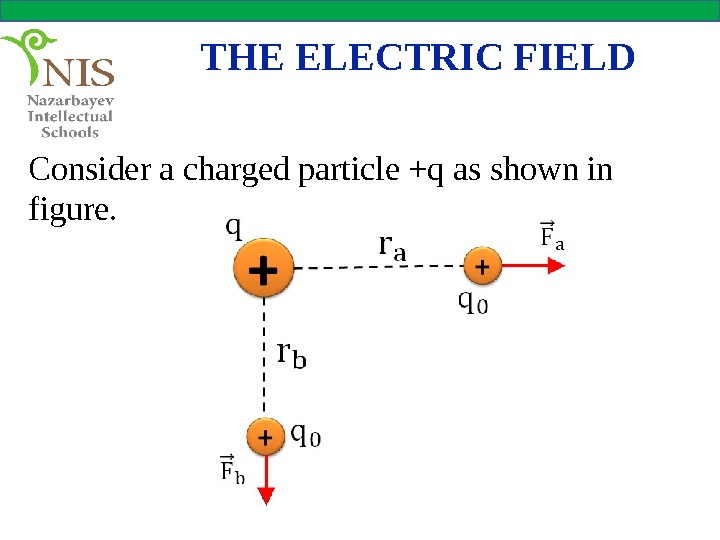THE ELECTRIC FIELD Consider a charged particle +q as shown in figure.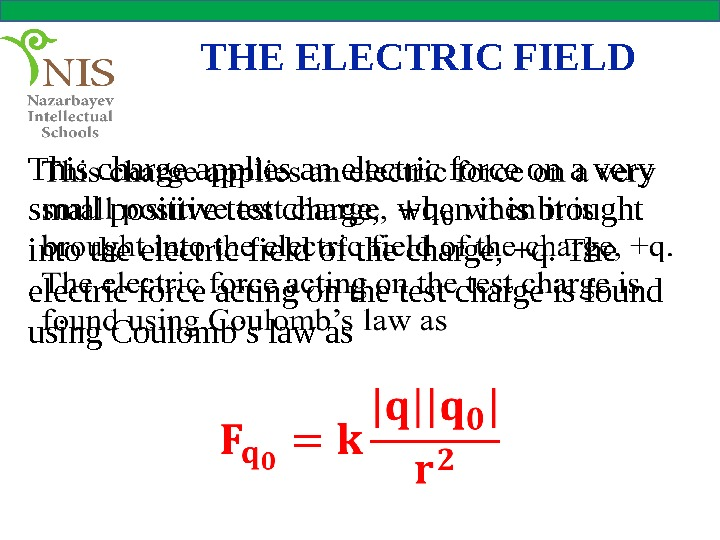THE ELECTRIC FIELD This charge applies an electric force on a very small positive test charge, when it is brought into the electric field of the charge, +q. The electric force acting on the test charge is found using Coulomb’s law as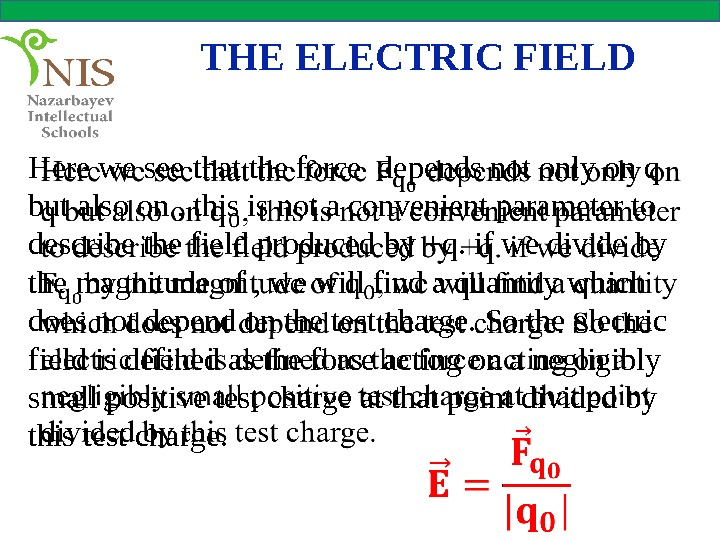THE ELECTRIC FIELD Here we see that the force depends not only on q but also on , this is not a convenient parameter to describe the field produced by +q. if we divide by the magnitude of , we will find a quantity which does not depend on the test charge. So the electric field is defined as the force acting on a negligibly small positive test charge at that point divided by this test charge.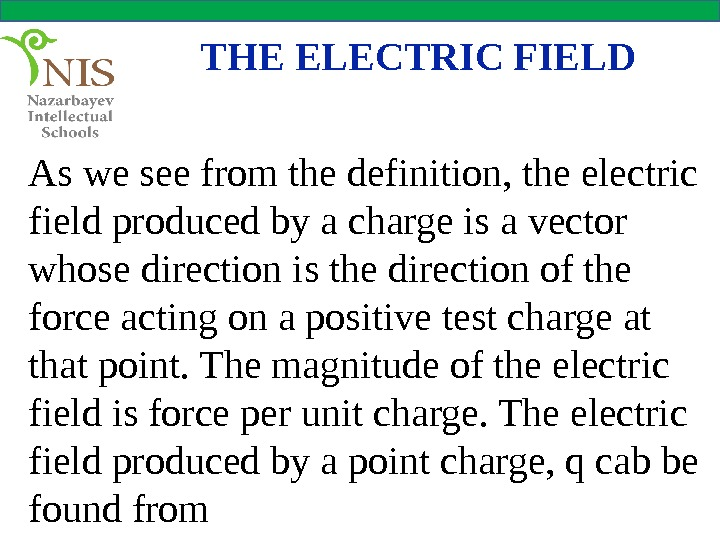THE ELECTRIC FIELD As we see from the definition, the electric field produced by a charge is a vector whose direction is the direction of the force acting on a positive test charge at that point. The magnitude of the electric field is force per unit charge. The electric field produced by a point charge, q cab be found from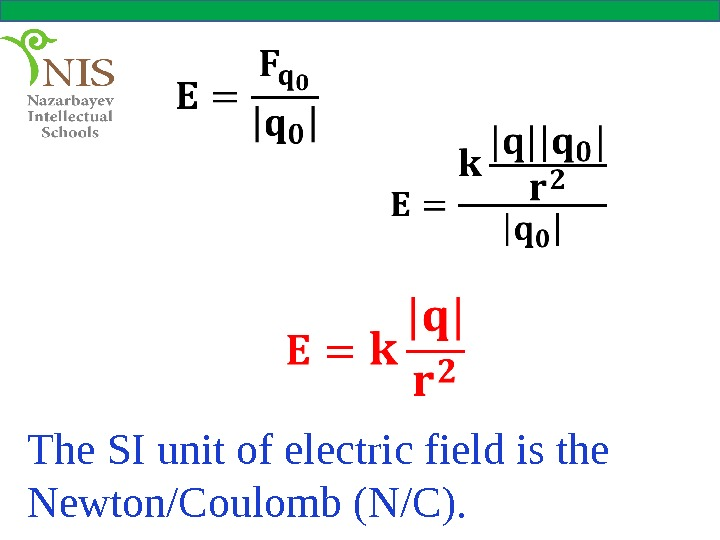The SI unit of electric field is the Newton/Coulomb (N/C).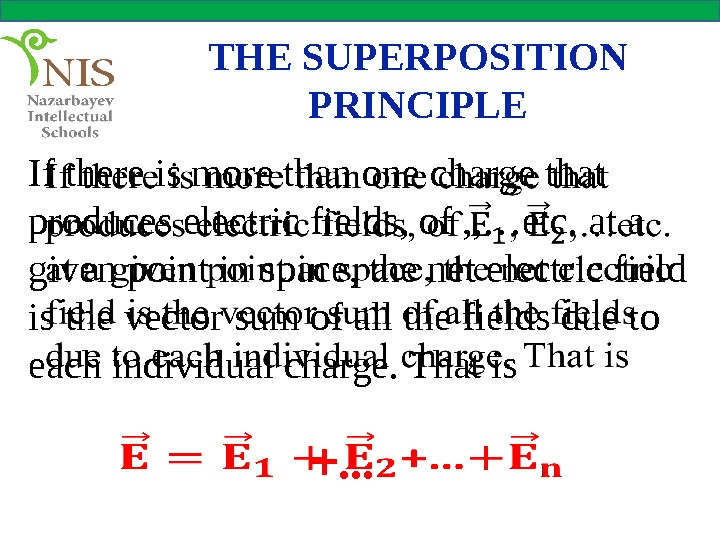THE SUPERPOSITION PRINCIPLE If there is more than one charge that produces electric fields, of , , …etc. at a given point in space, the net electric field is the vector sum of all the fields due to each individual charge. That is +…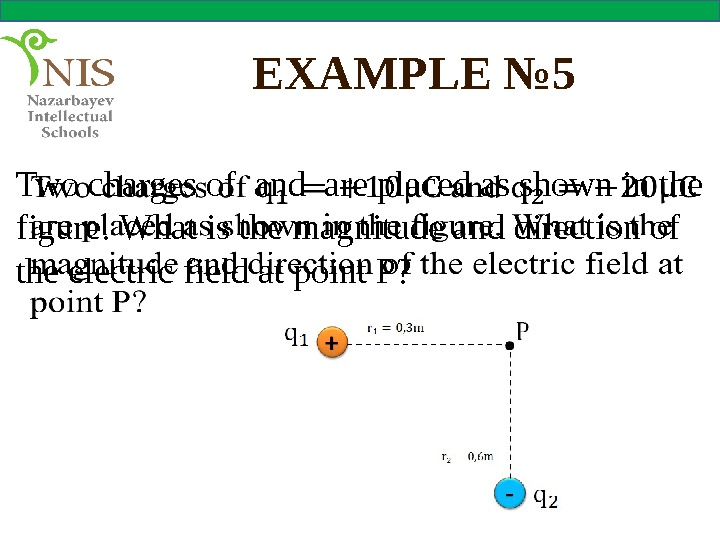EXAMPLE № 5 Two charges of and are placed as shown in the figure. What is the magnitude and direction of the electric field at point P?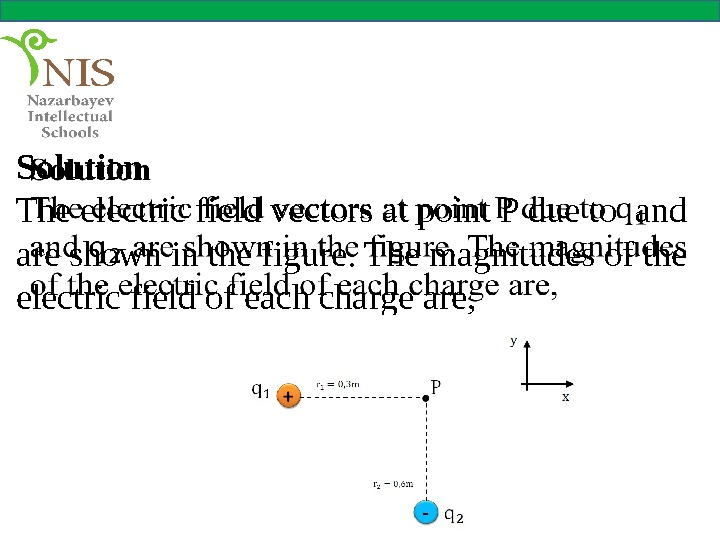Solution The electric field vectors at point P due to and are shown in the figure. The magnitudes of the electric field of each charge are,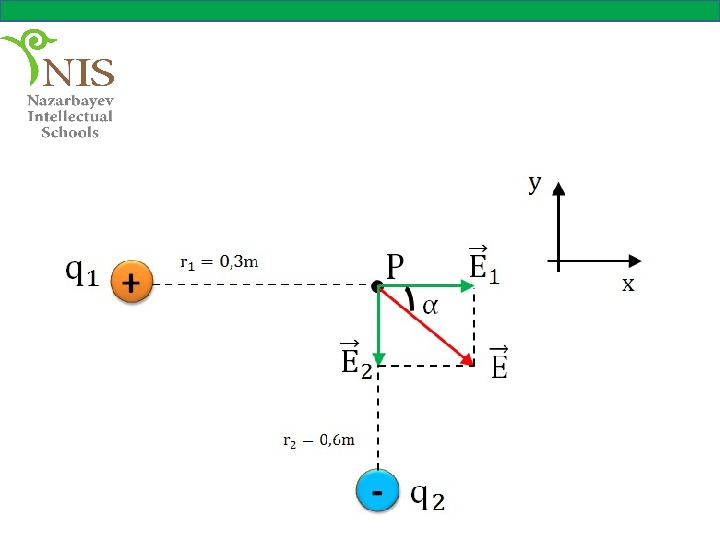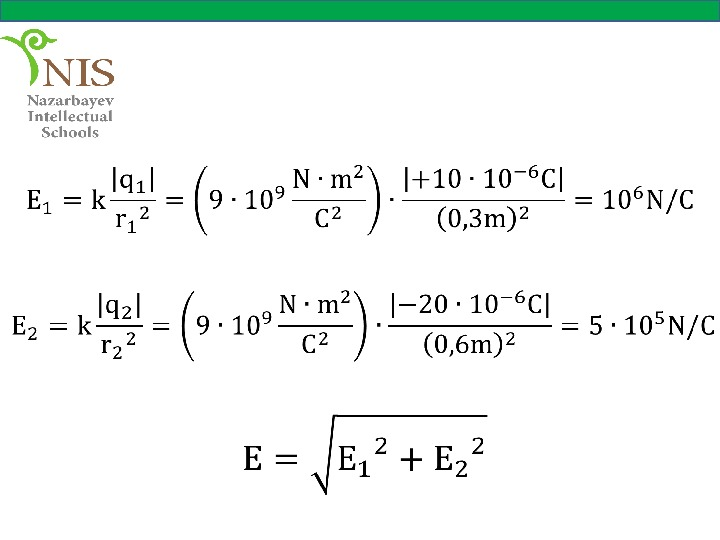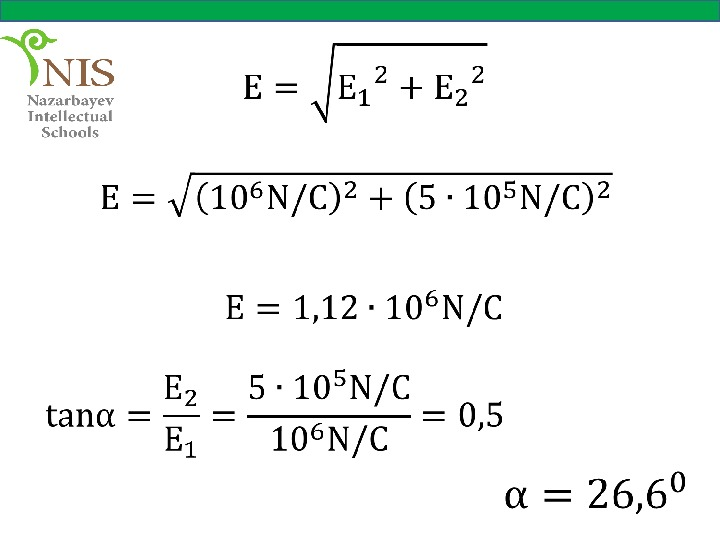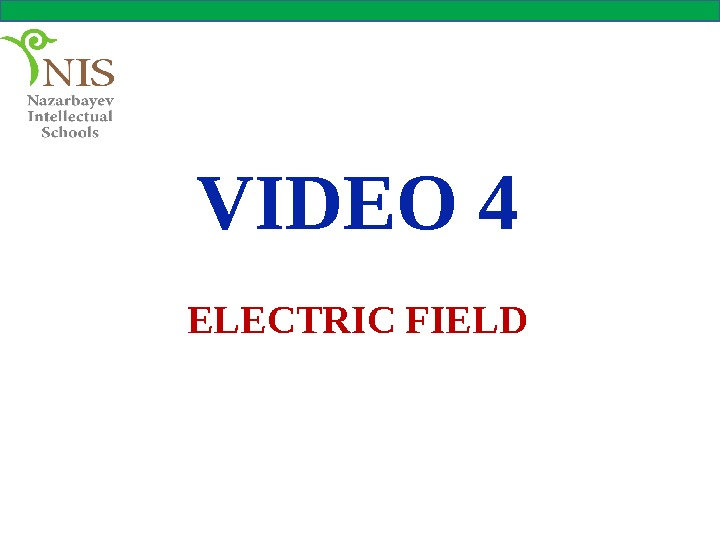VIDEO 4 ELECTRIC FIEL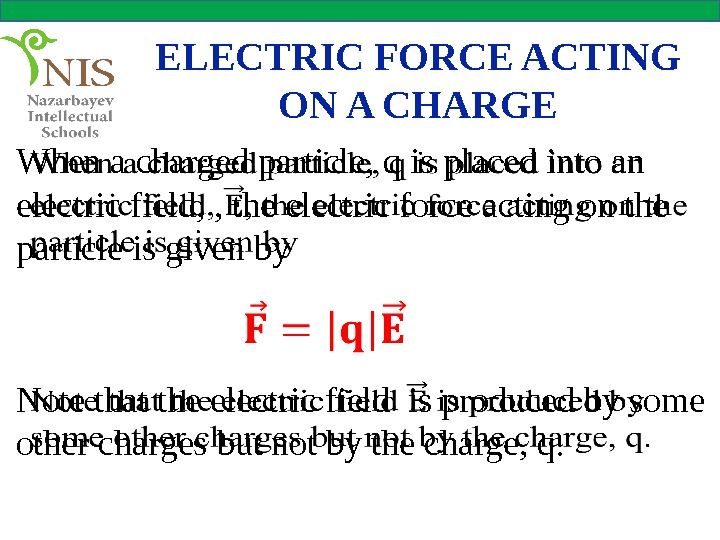ELECTRIC FORCE ACTING ON A CHARGE When a charged particle, q is placed into an electric field, , the electric force acting on the particle is given by Note that the electric field is produced by some other charges but not by the charge, q.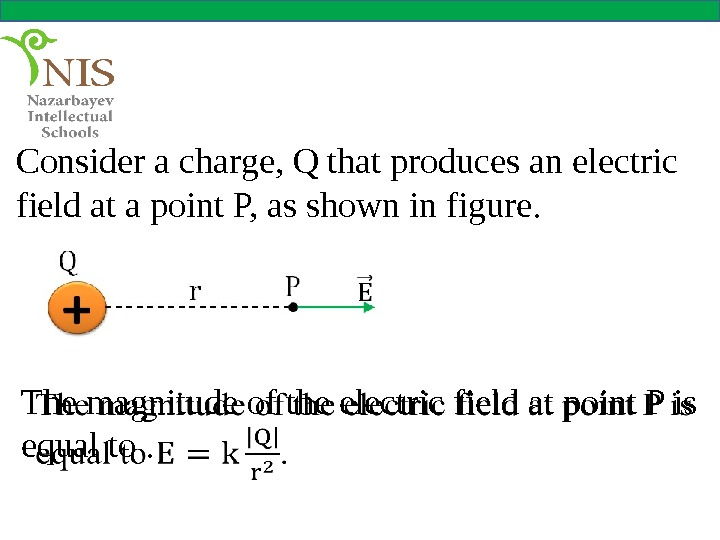Consider a charge, Q that produces an electric field at a point P, as shown in figure. The magnitude of the electric field at point P is equal to.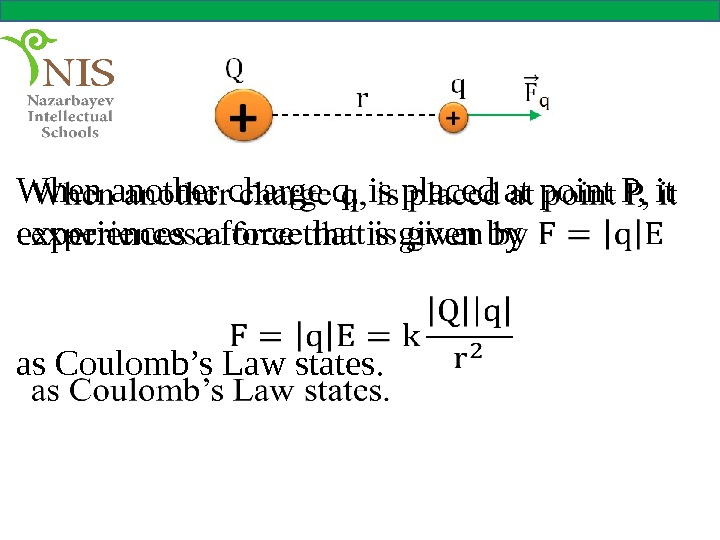When another charge q, is placed at point P, it experiences a force that is given by as Coulomb’s Law states.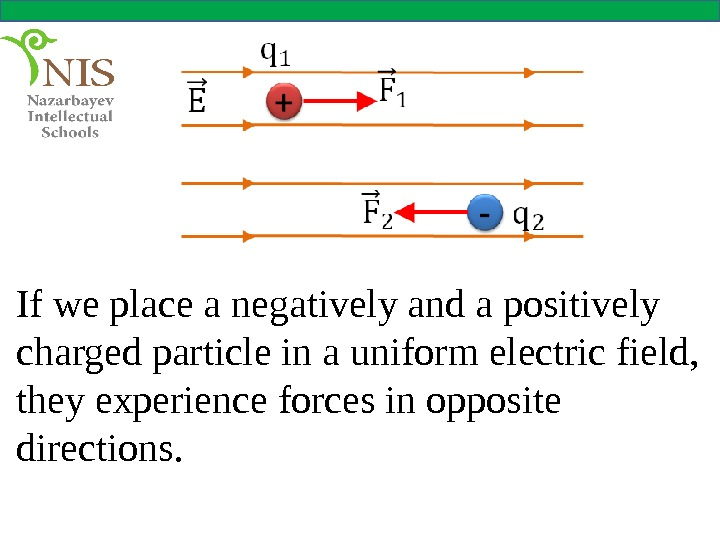If we place a negatively and a positively charged particle in a uniform electric field, they experience forces in opposite directions.INFORMATION ABOUT LESSON AND TEST BANK YOU CAN GET ON http: //moodle. nis. edu. kzHOMEWORK physics nis kokshetau @mail. ru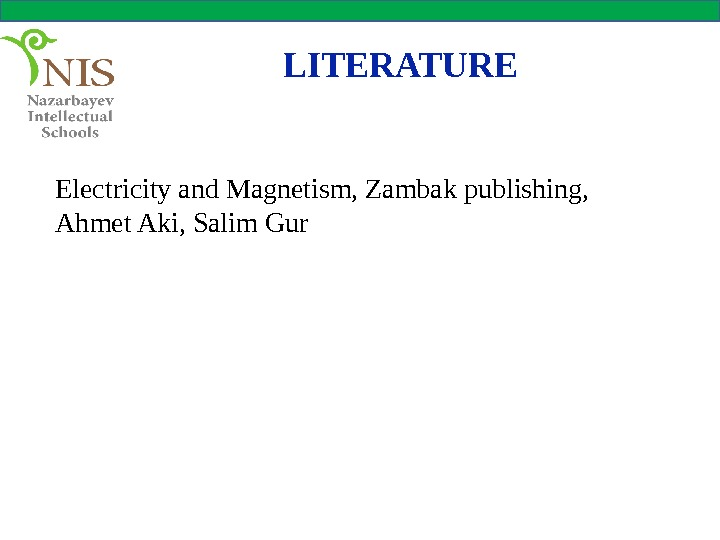LITERATURE Electricity and Magnetism, Zambak publishing, Ahmet Aki, Salim GurTHANK YOU FOR YOUR ATTENTION